# Madhya Pradesh Board Question Papers for Class 12th Maths 2020 With Solutions In PDF

Madhya Pradesh Class 12 Maths 2020 question paper with solutions are provided here in a downloadable pdf format and also in the text, so that the students have an idea of the Maths marking scheme. Along with the solutions, maths question paper 2020 Class 12 is also added here for reference.

MPBSE 12th Students will be able to access all the MPBSE 12th previous year maths question papers. The solutions provided by BYJU’S will encourage the students to practise well and prepare for the exam to complete the paper in a stipulated time, which enhances their writing speed with accuracy. It can be downloaded easily and in a shorter period of time without any hassle.

Solving 2020 Maths question papers and also the previous year papers for Class 12 will boost one’s preparation level by making them aware of the type of questions asked in the upcoming board exams.

### QUESTION PAPER CODE E – 823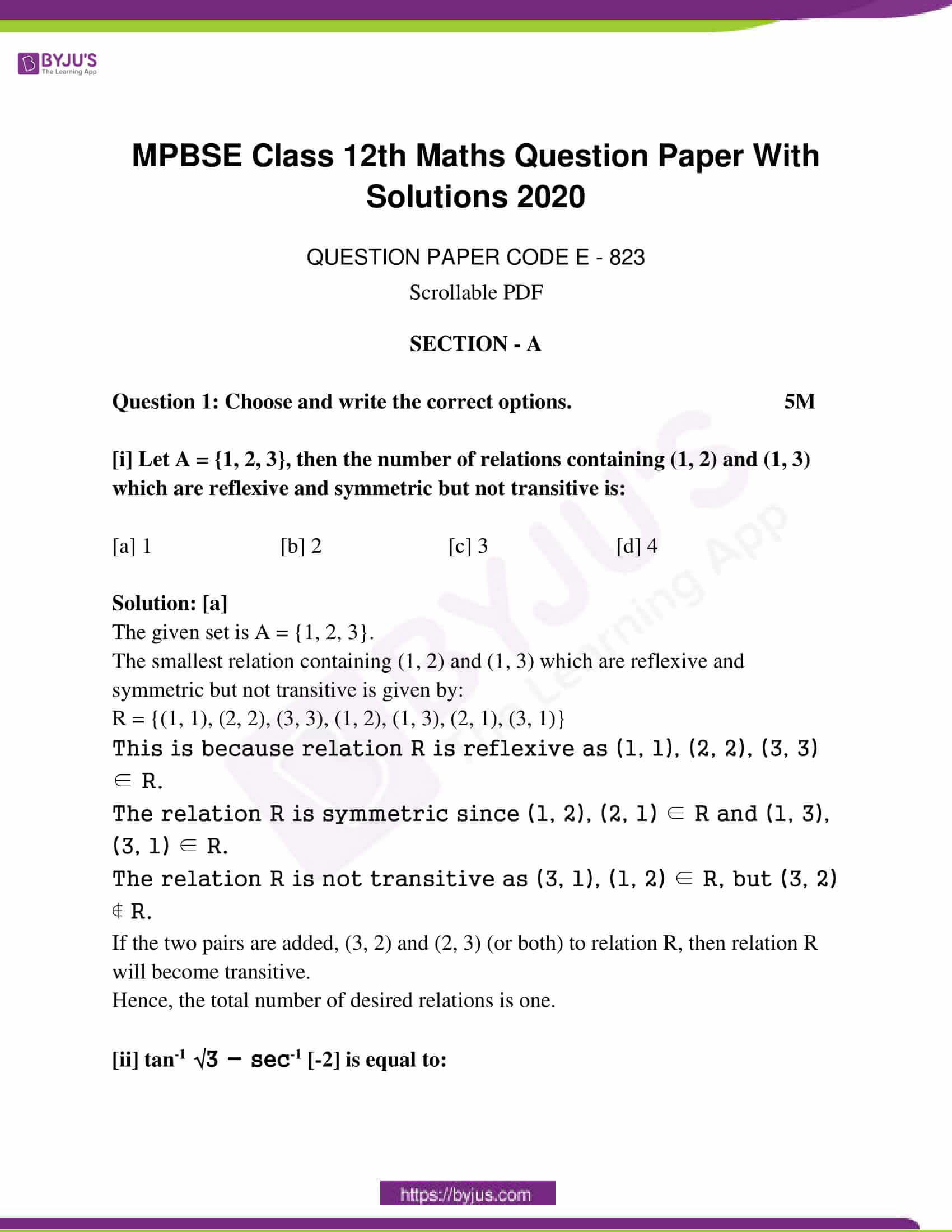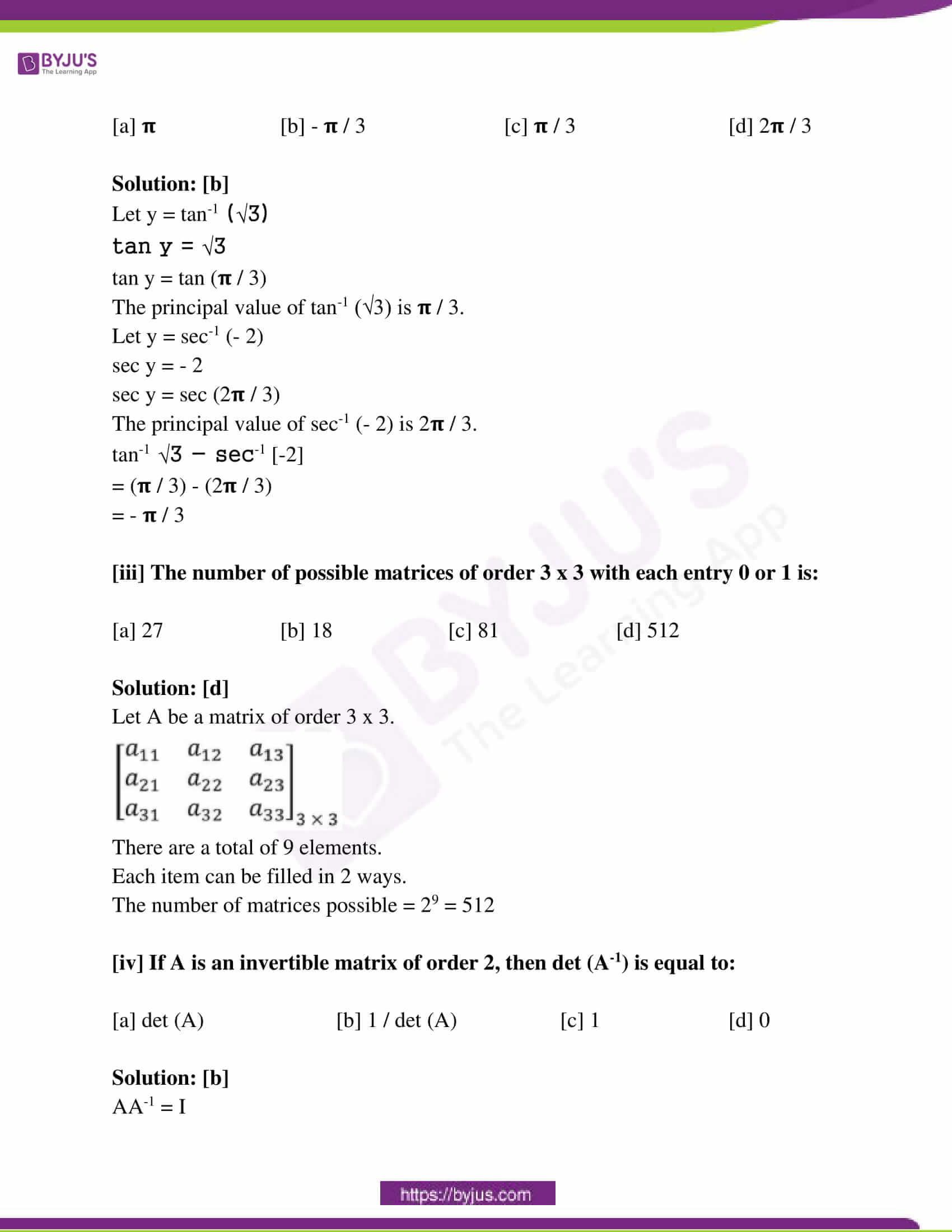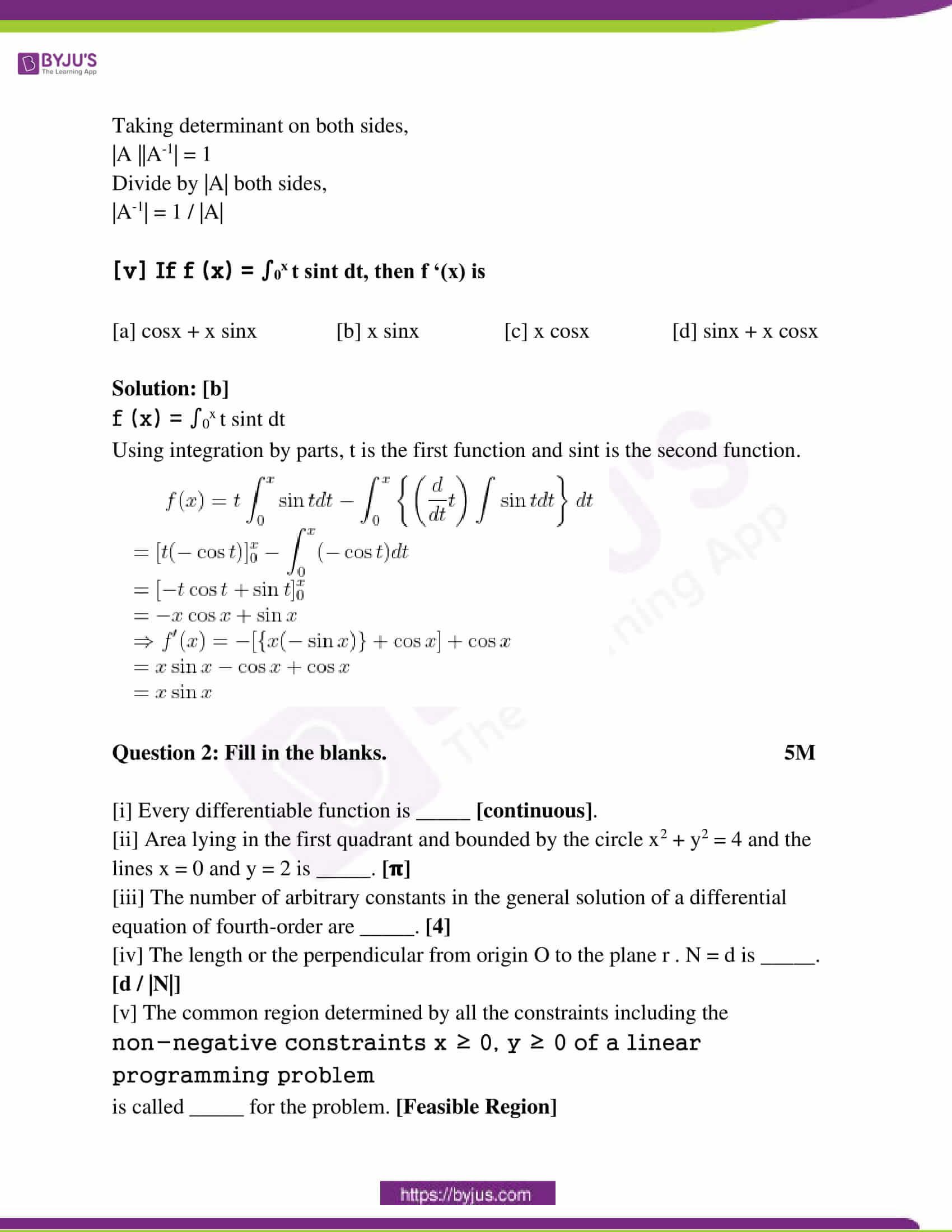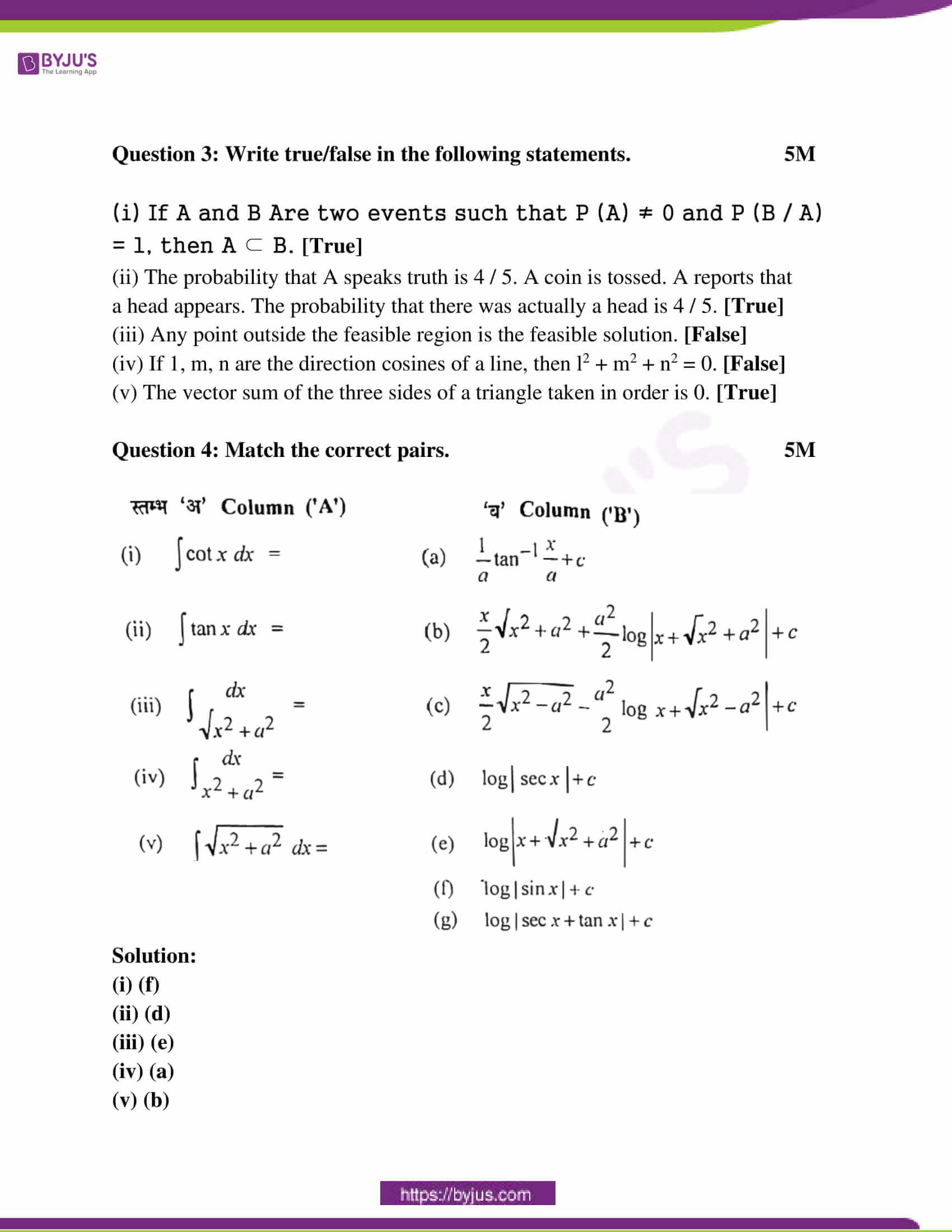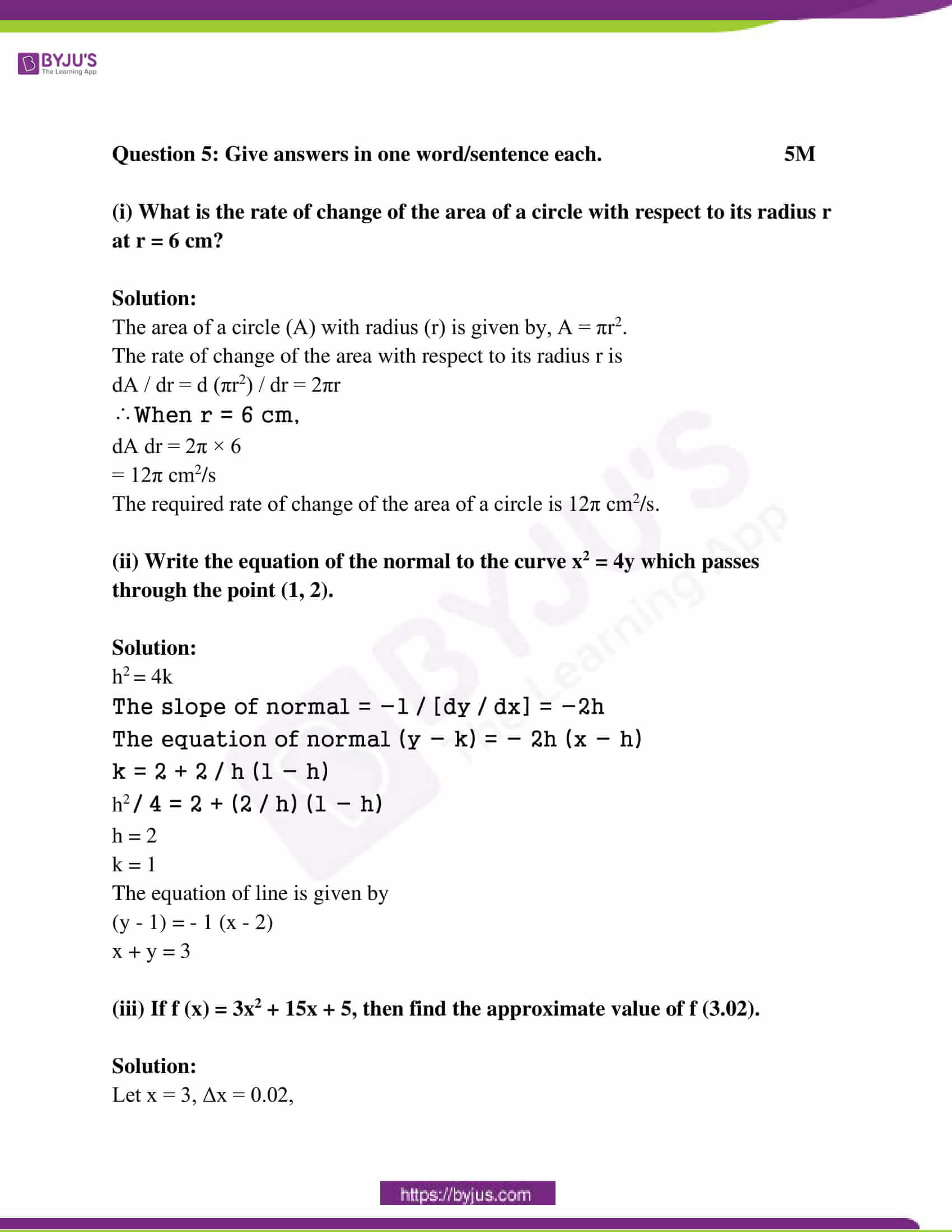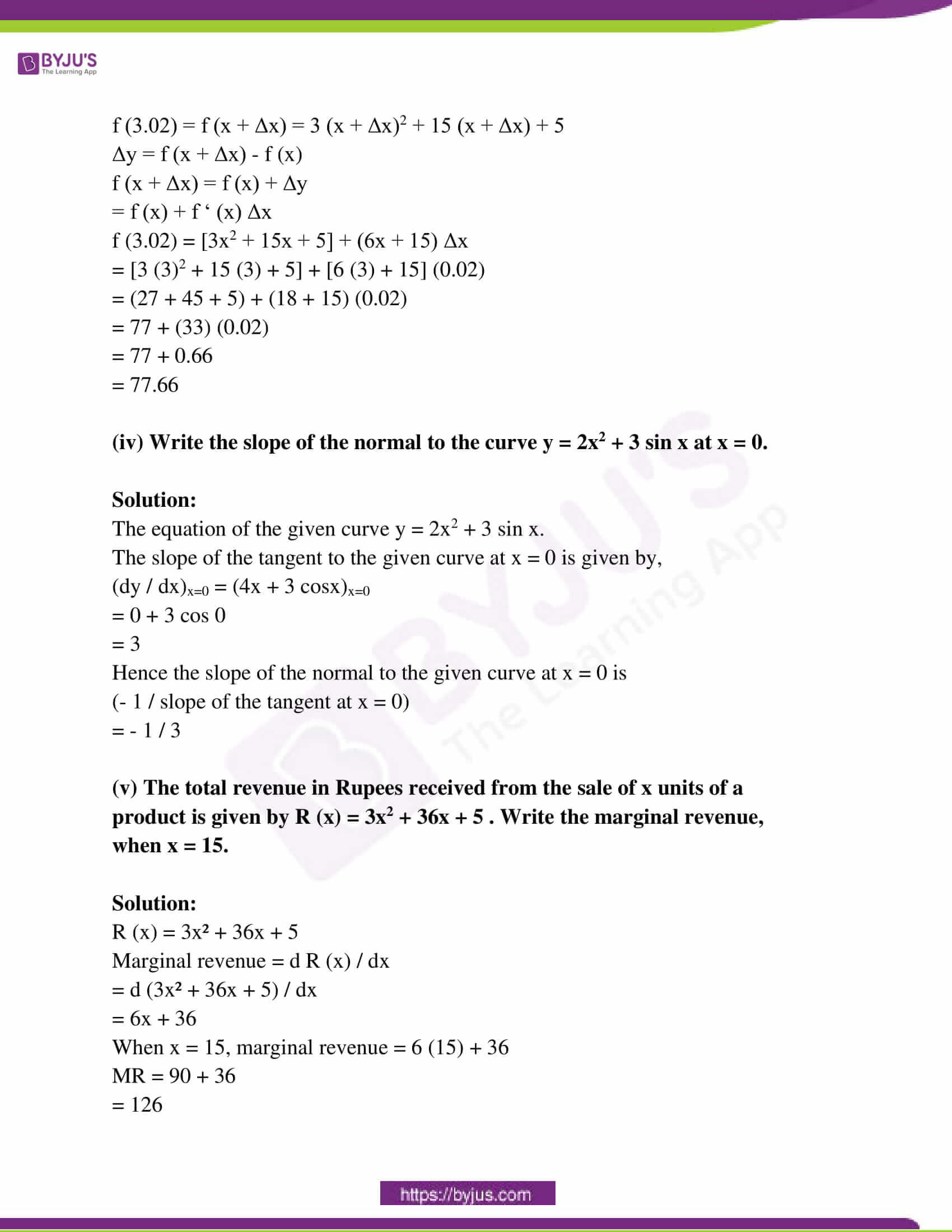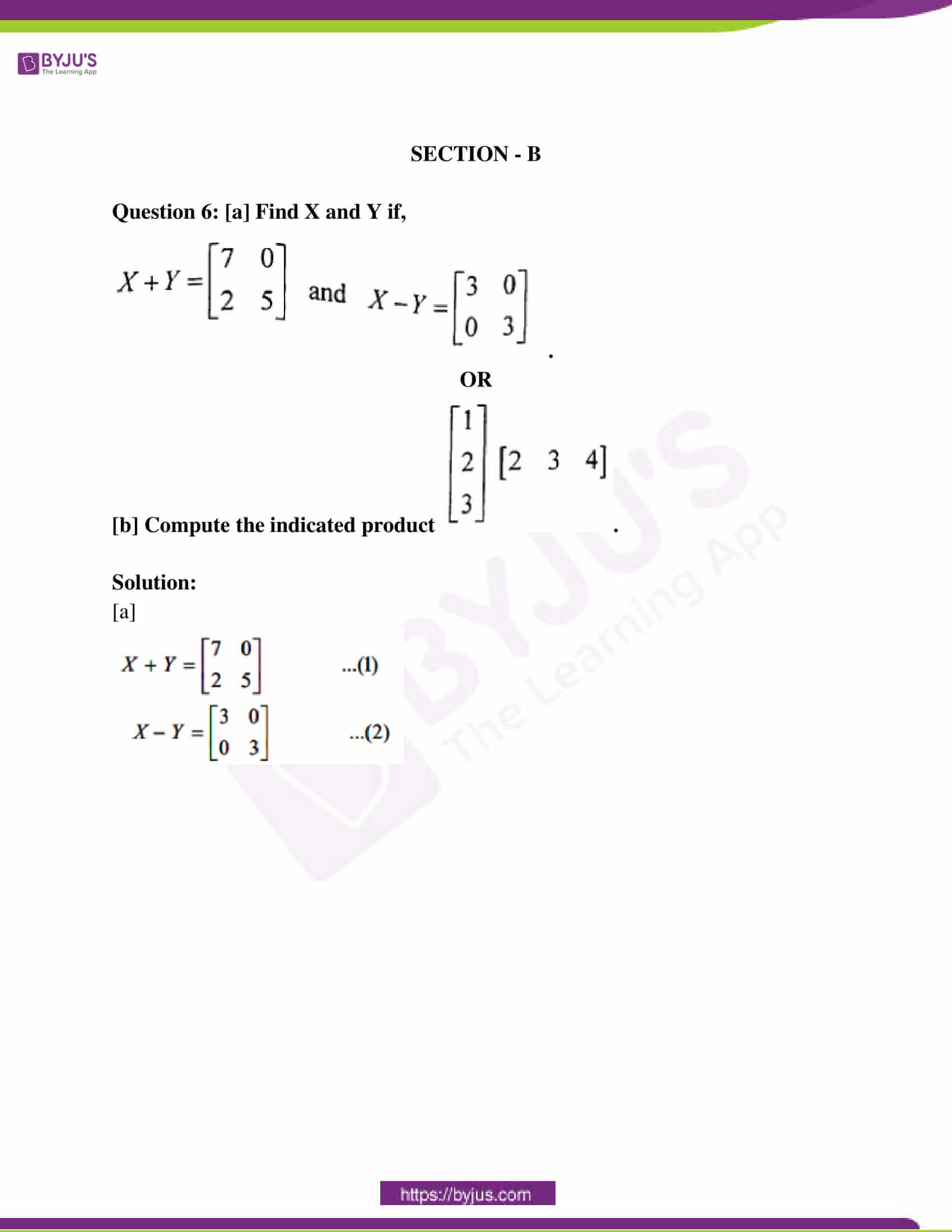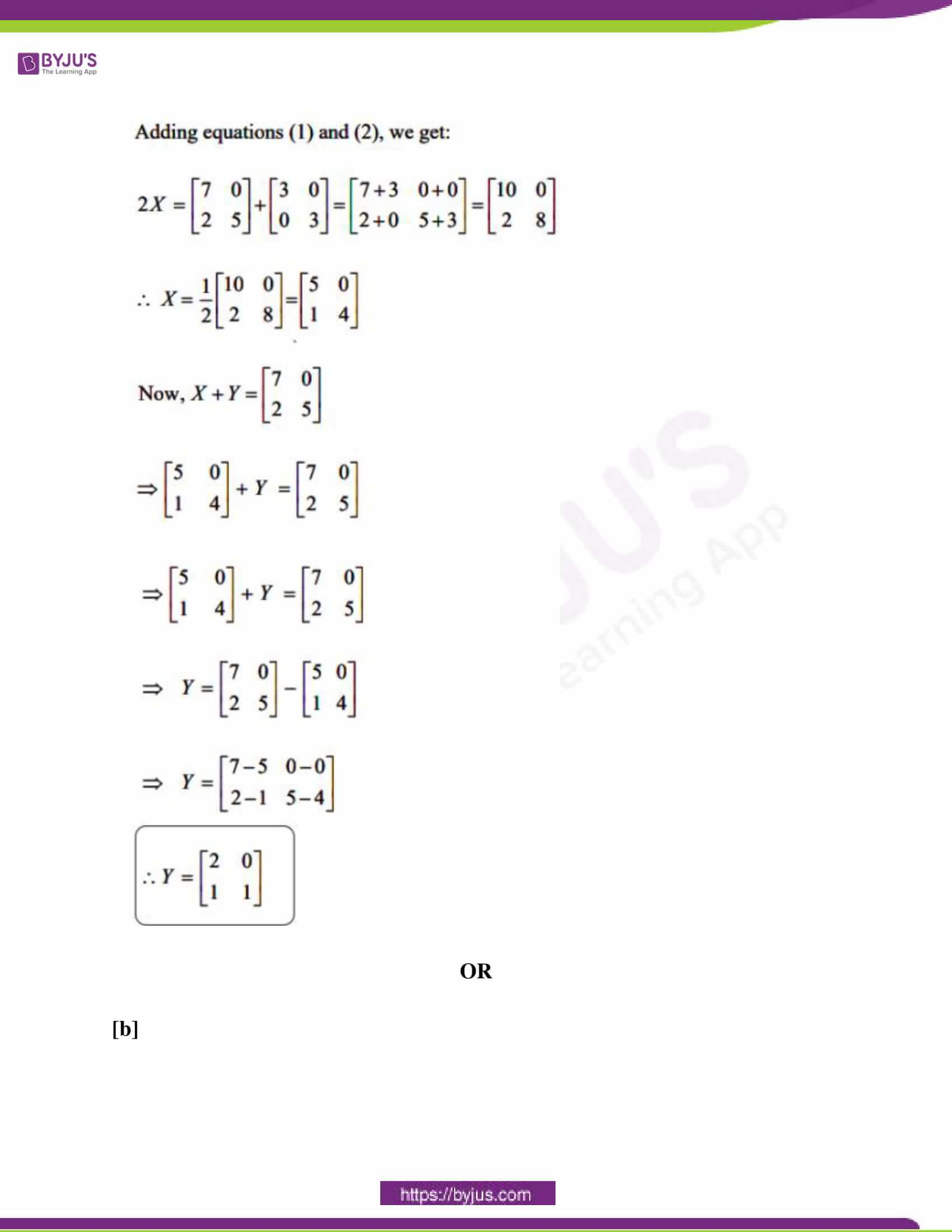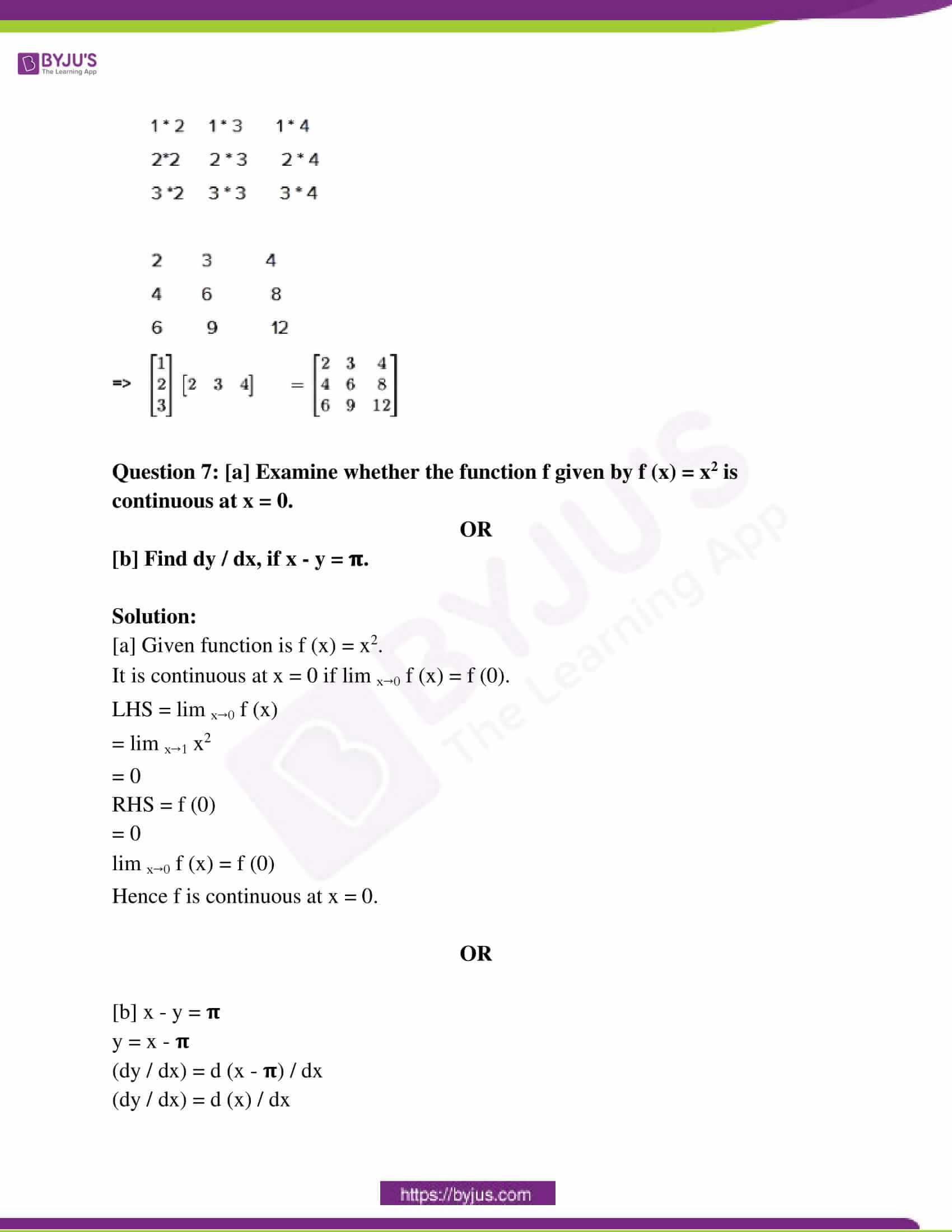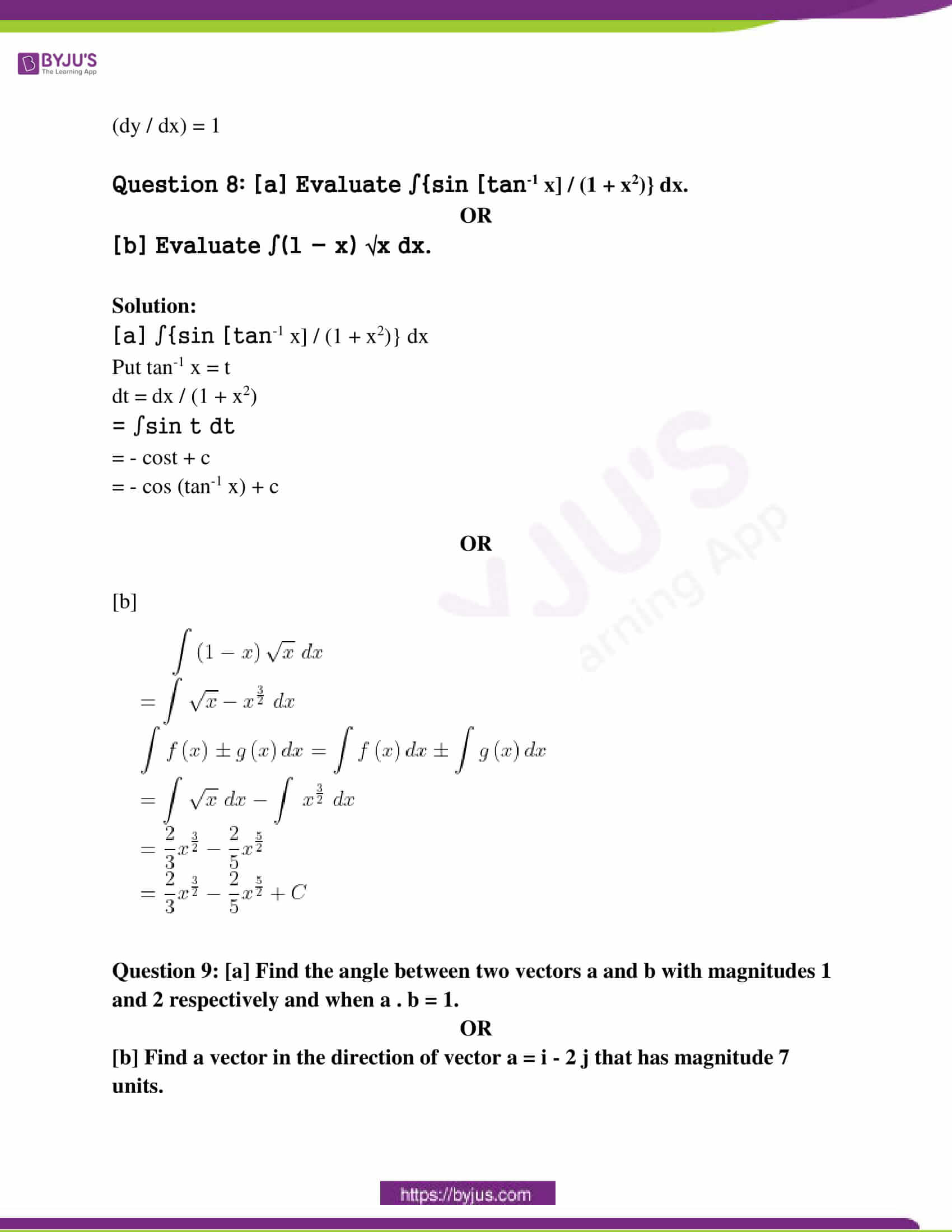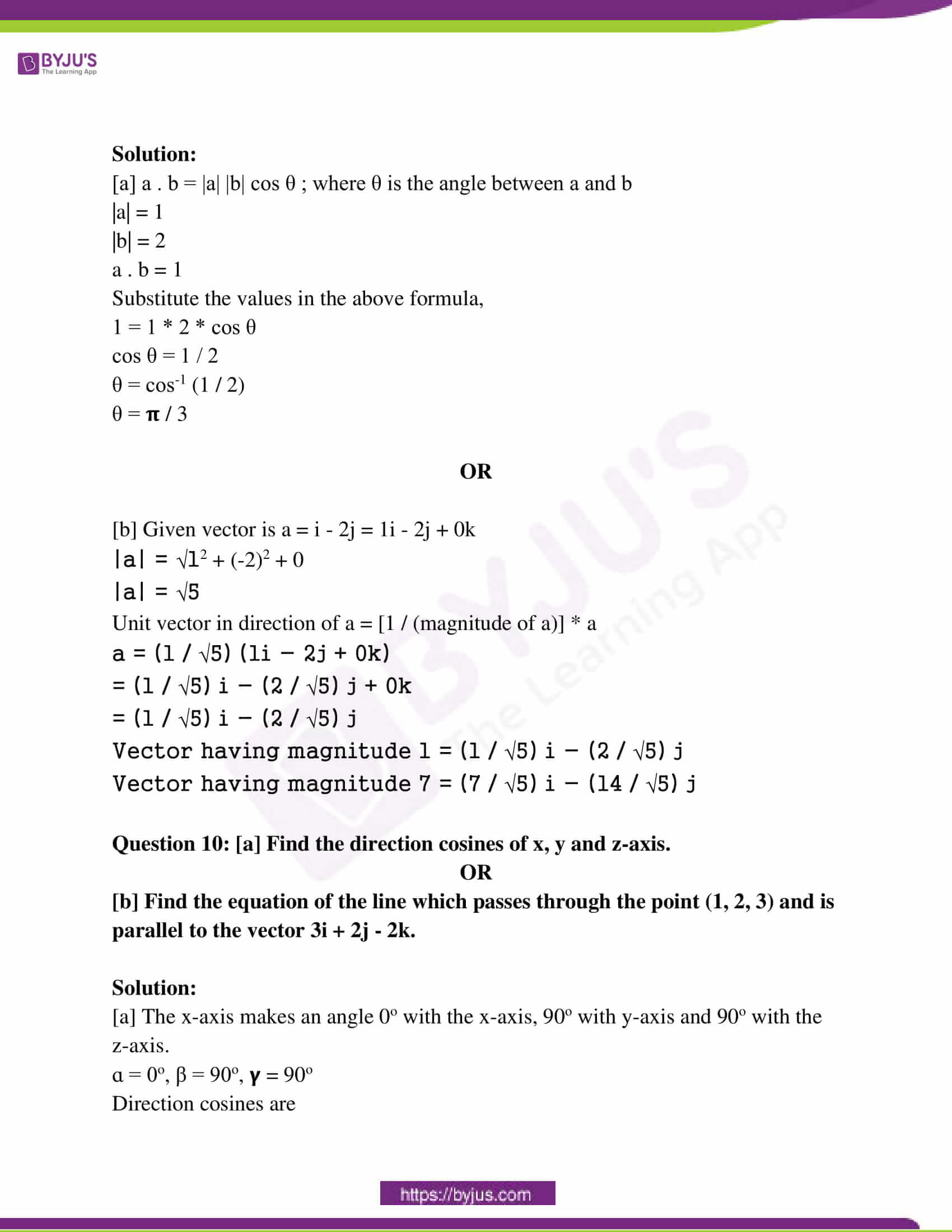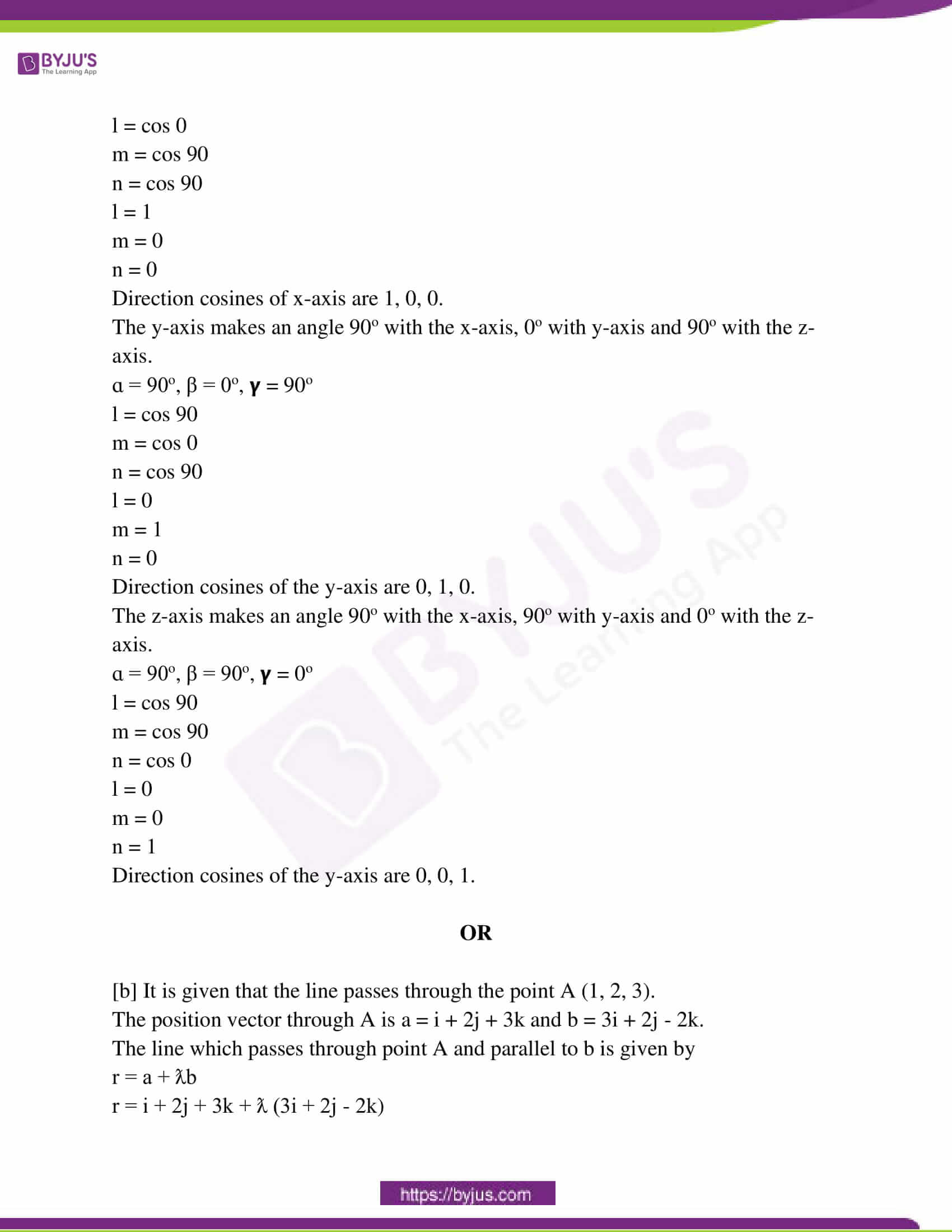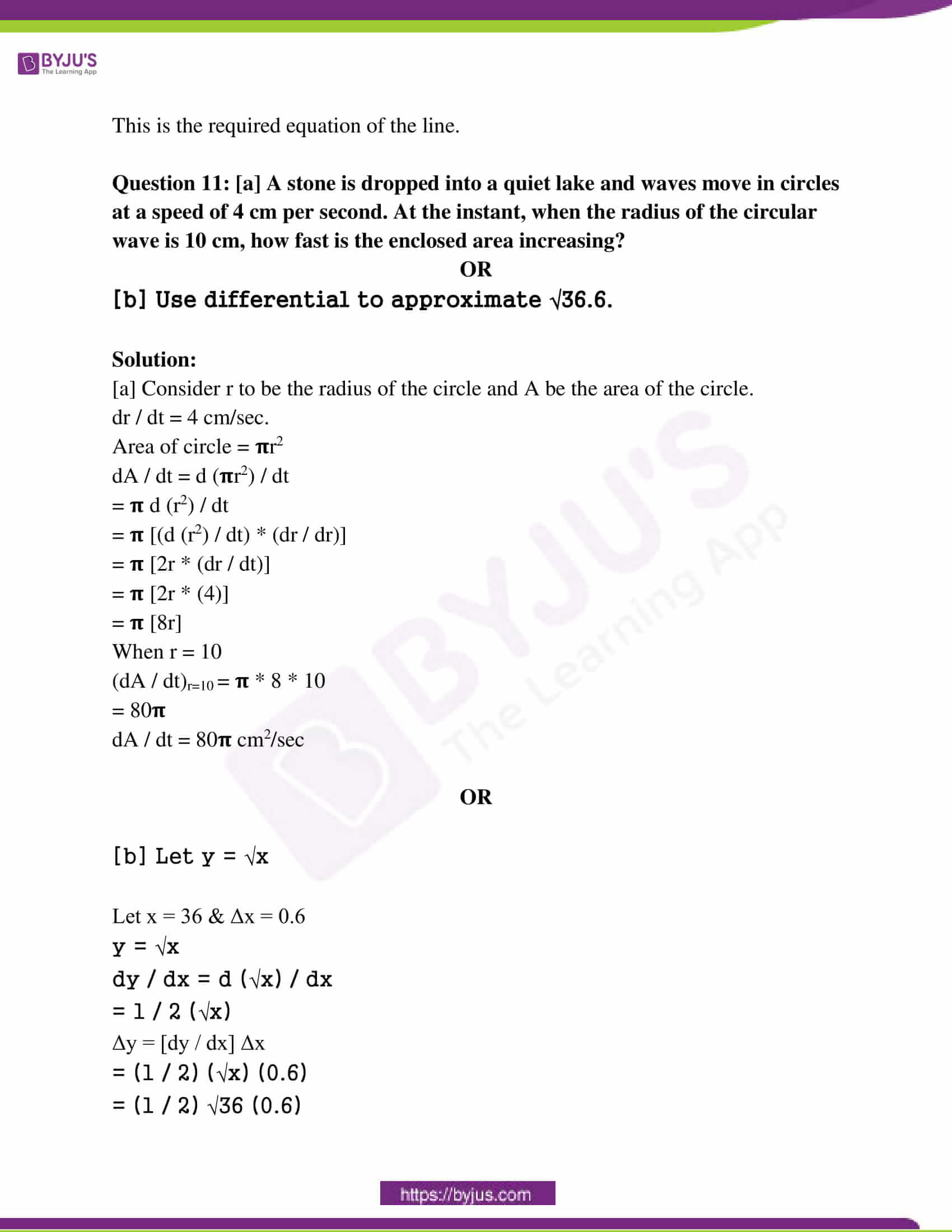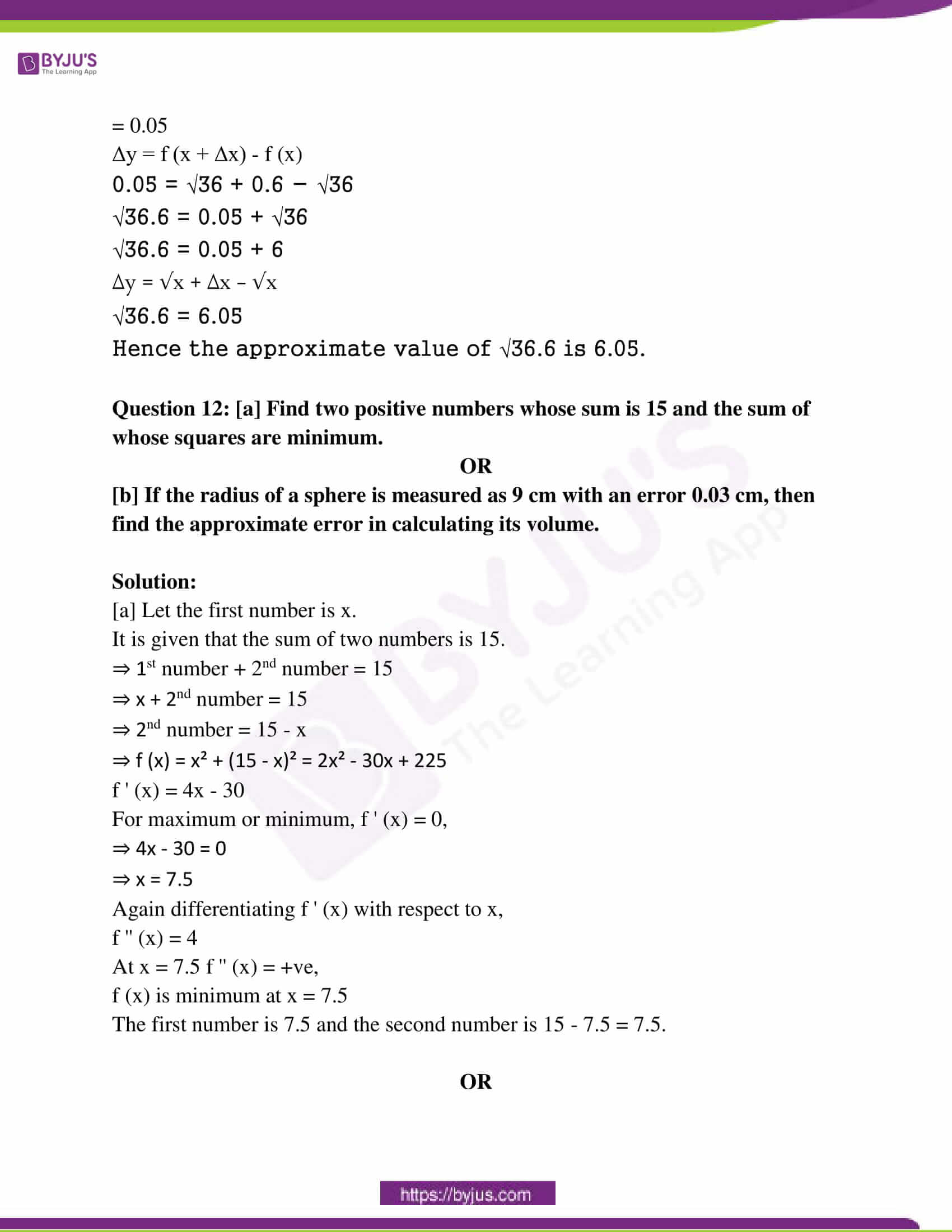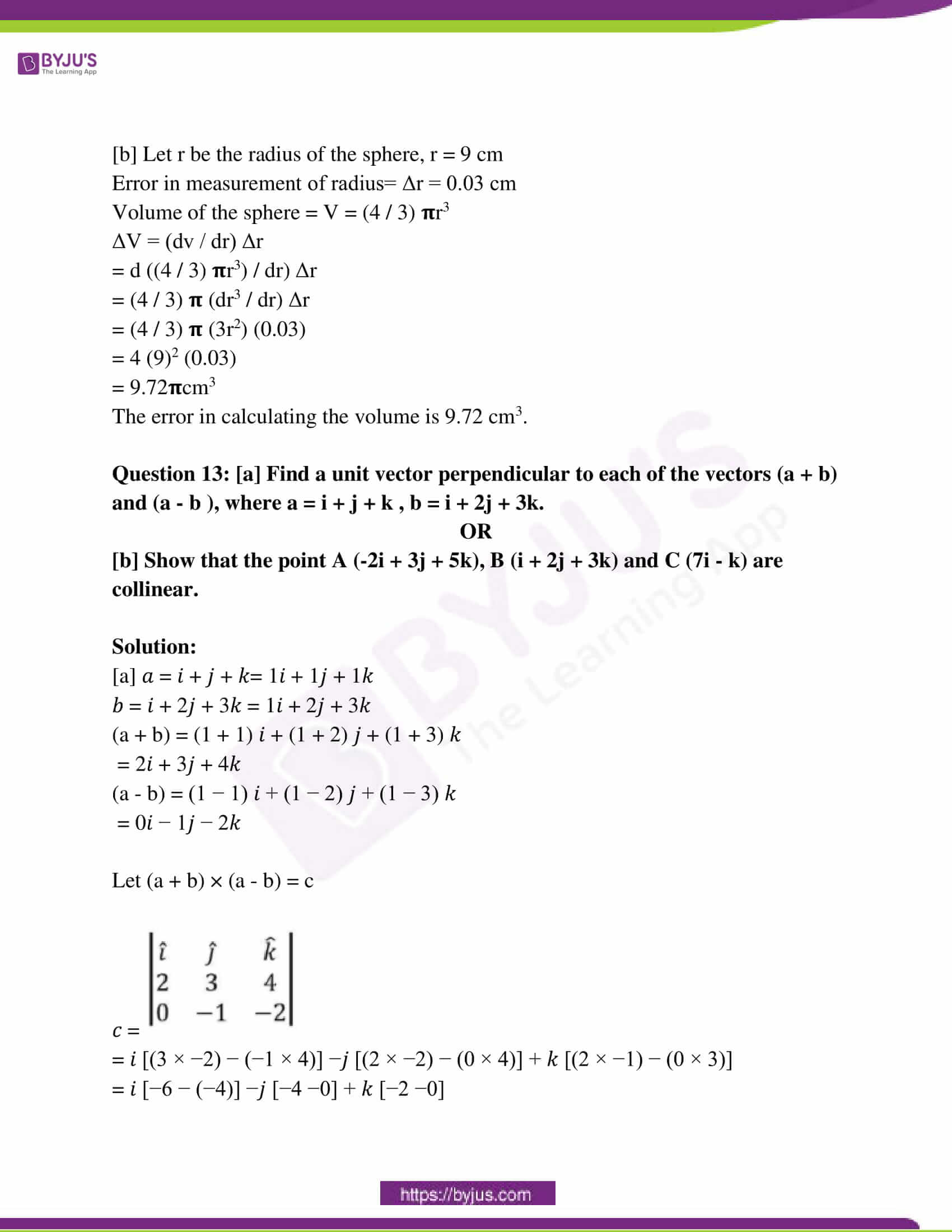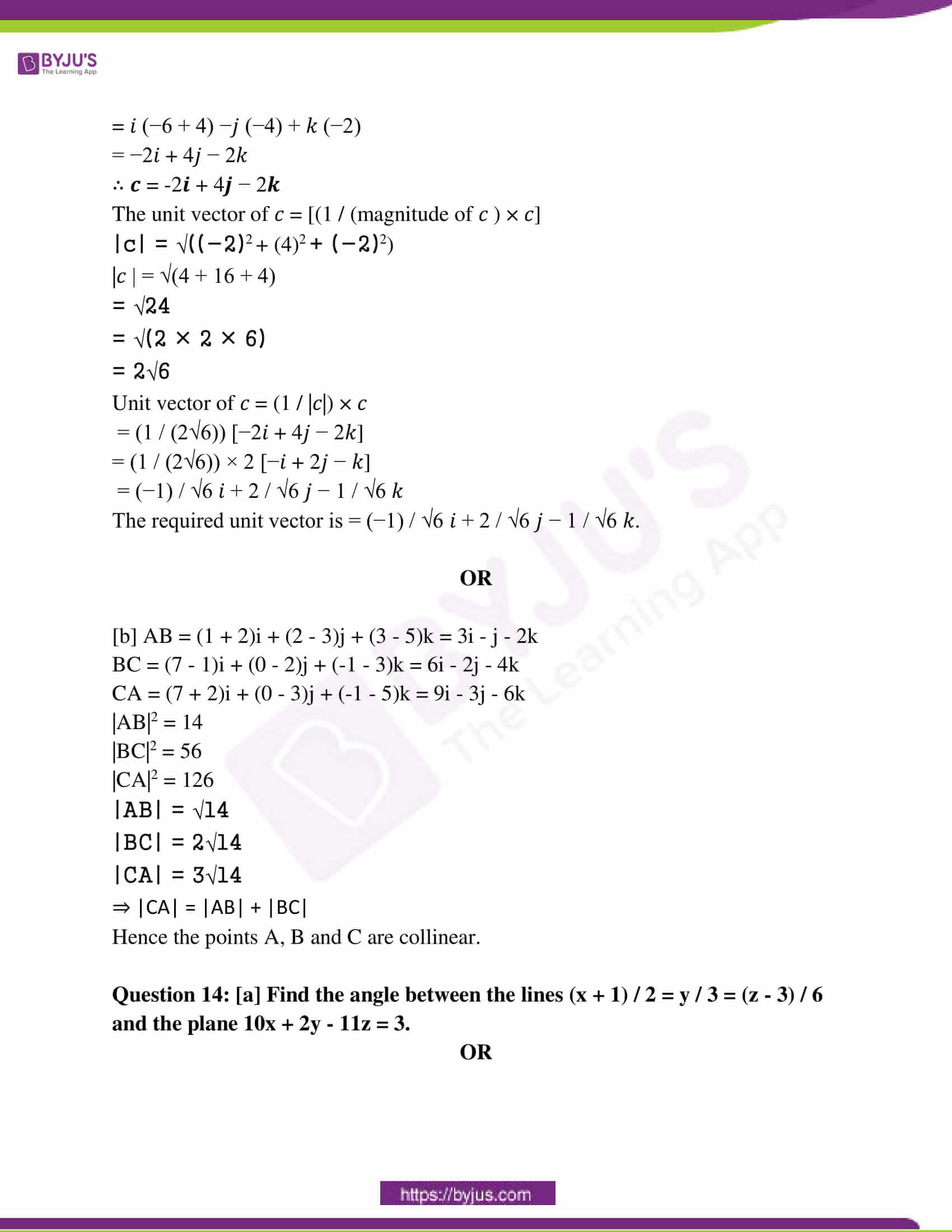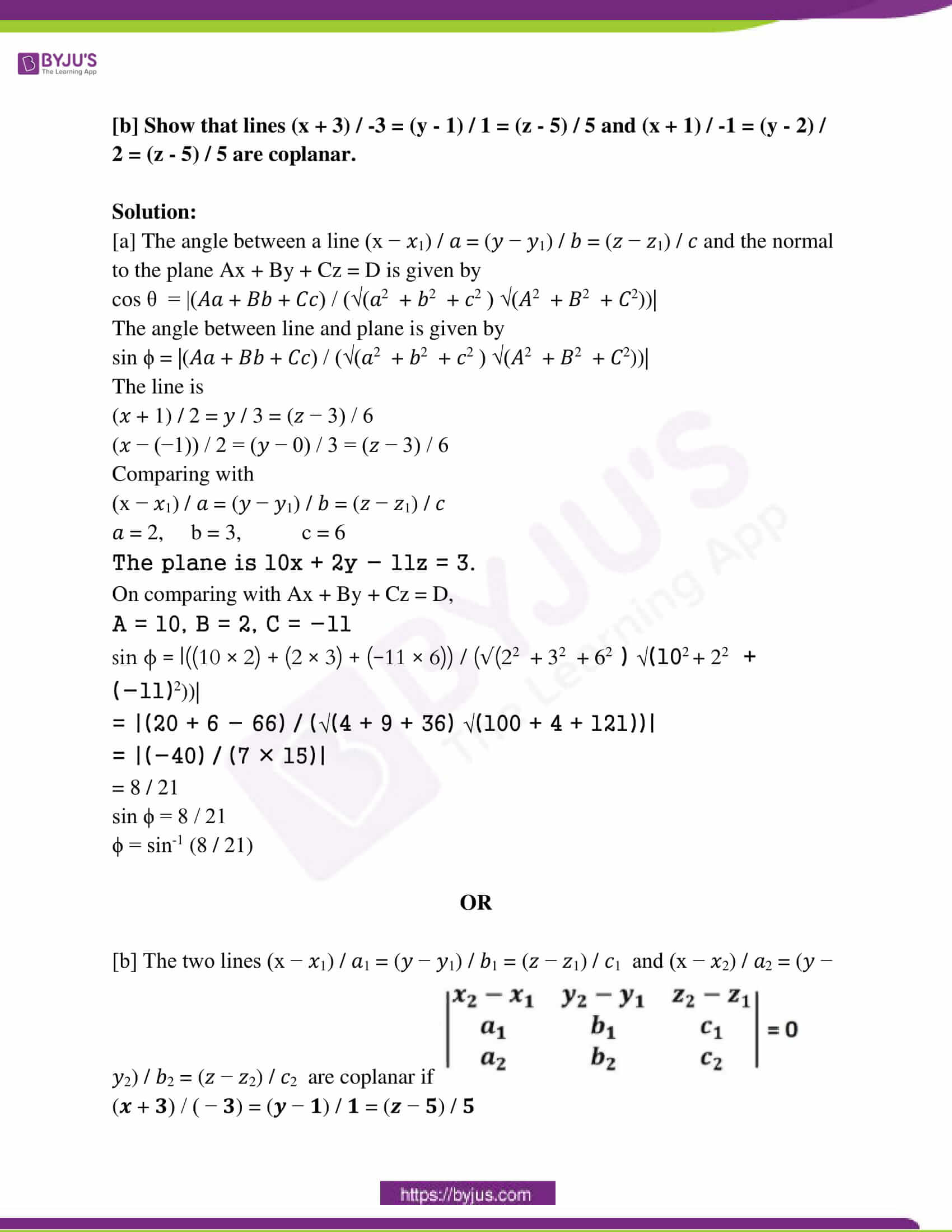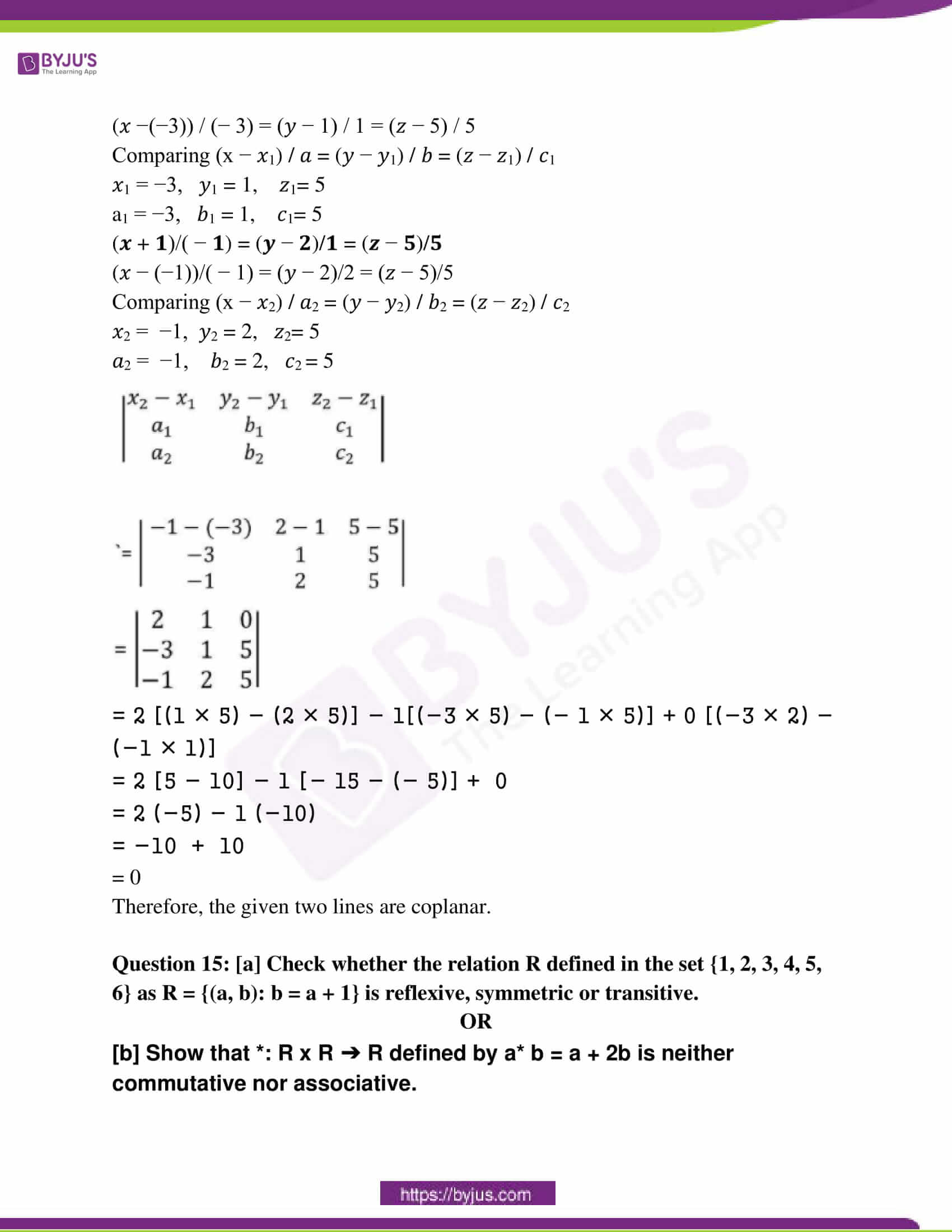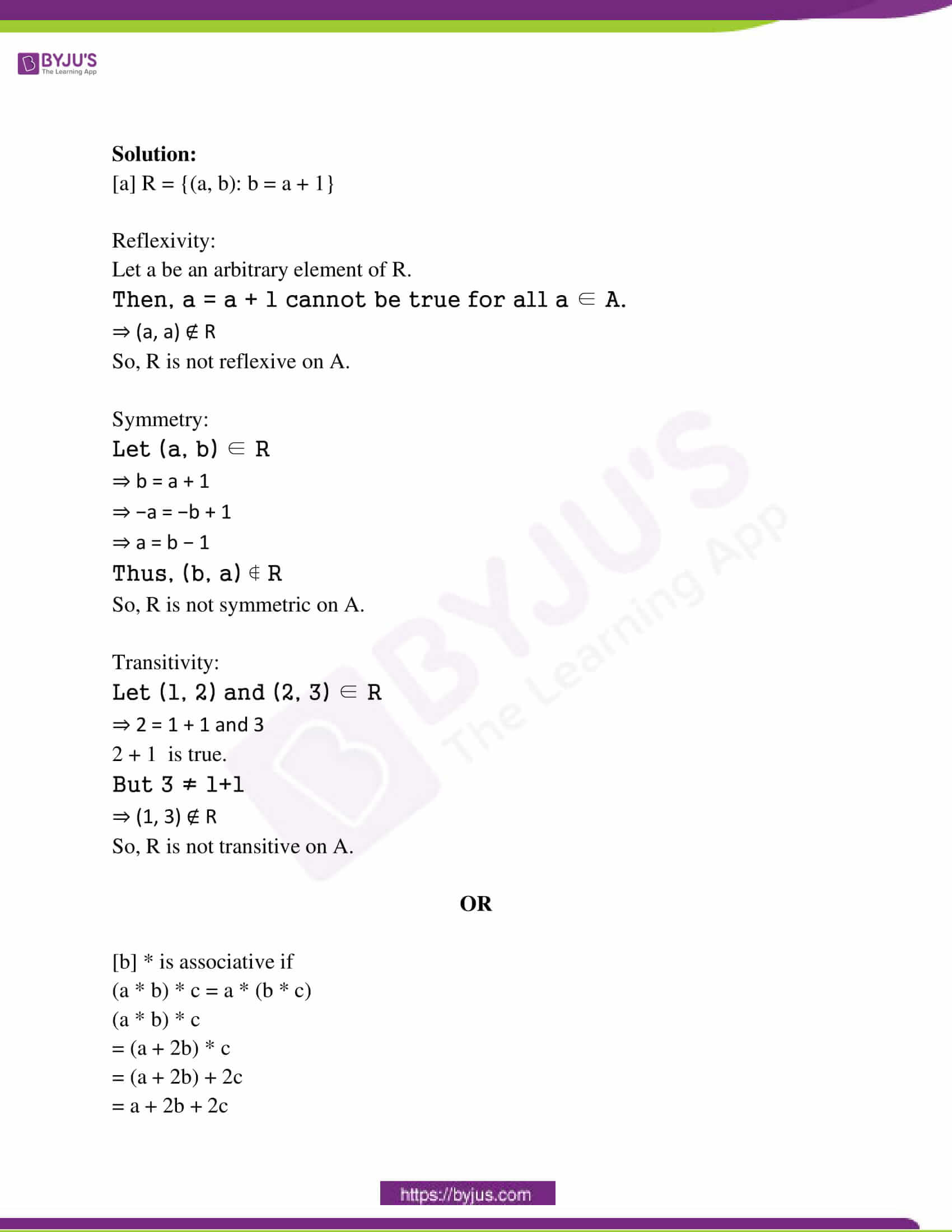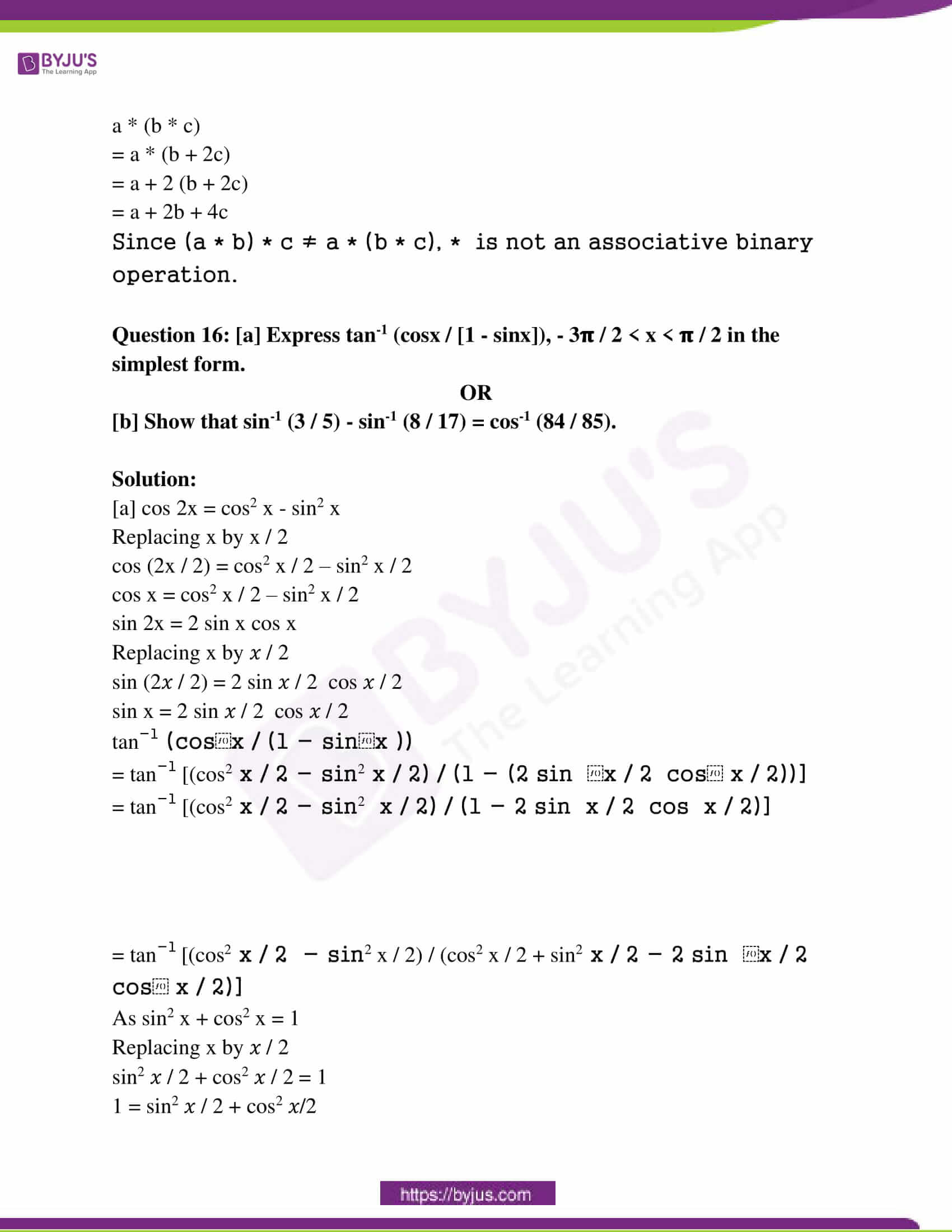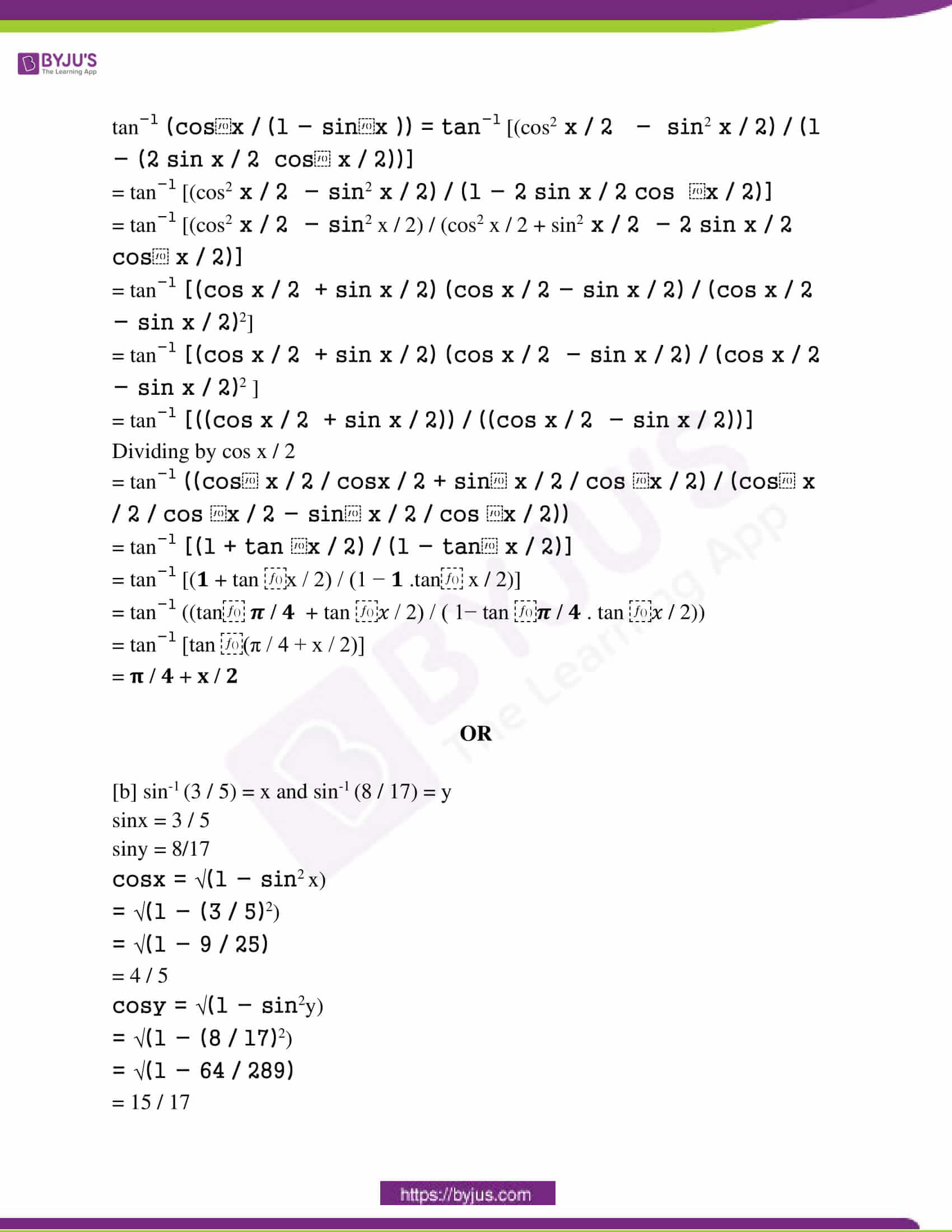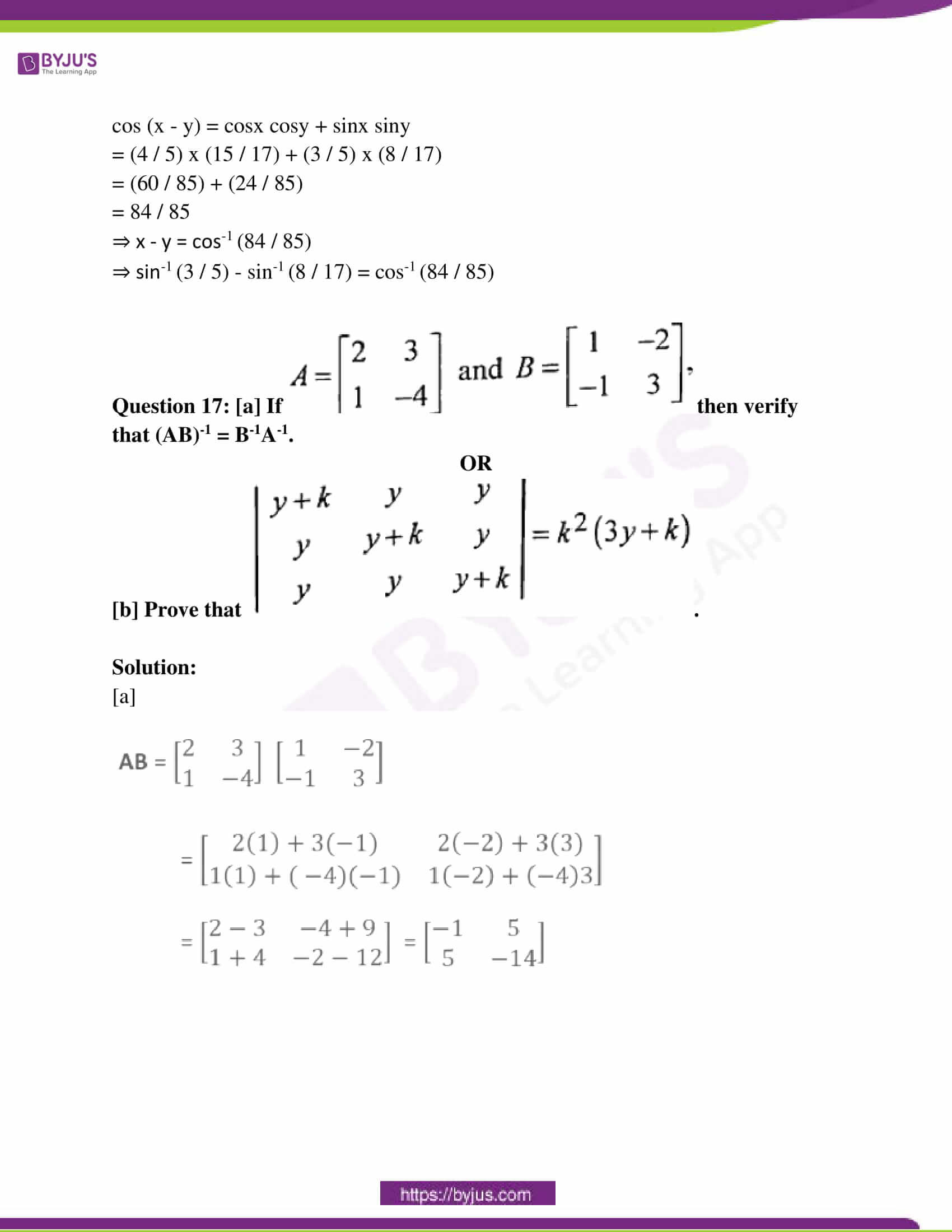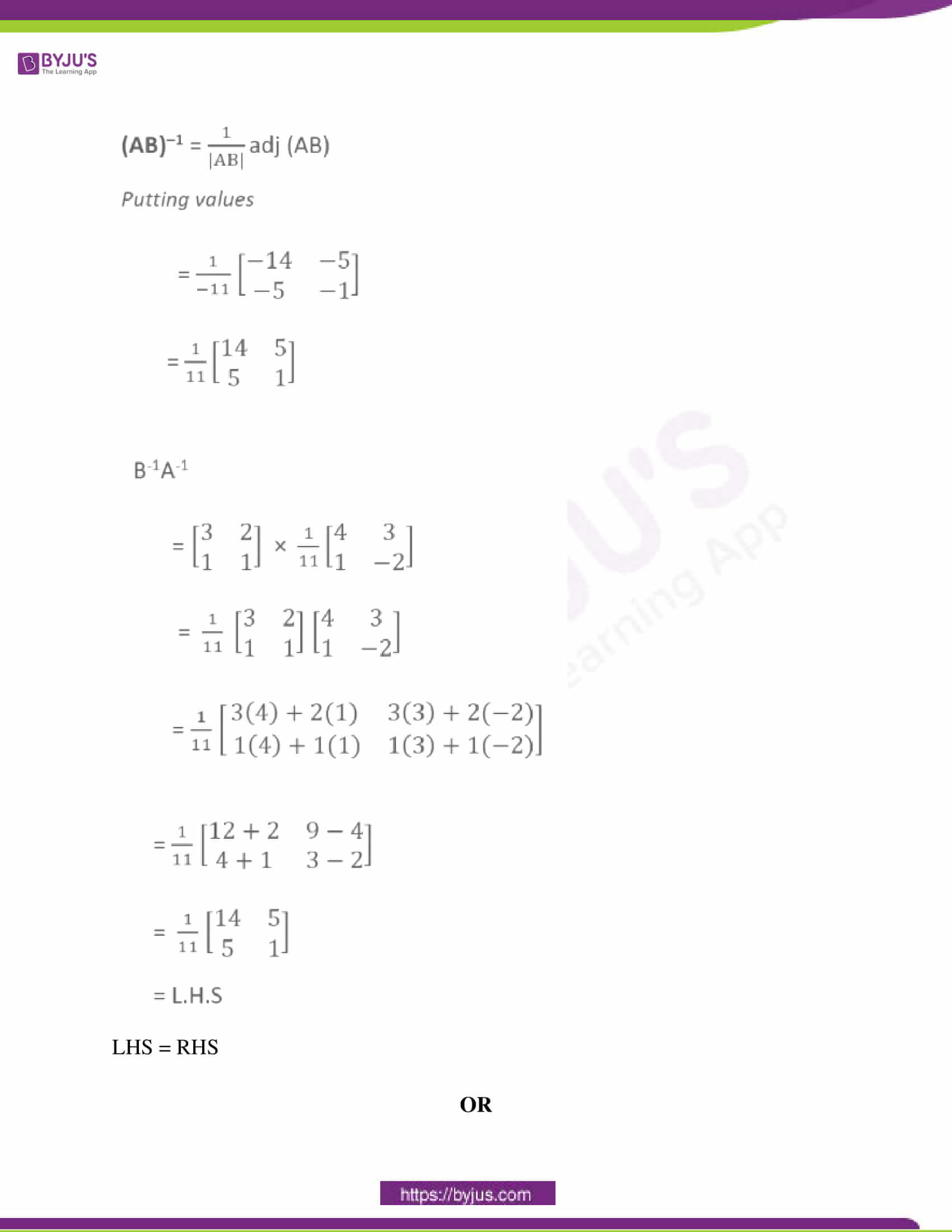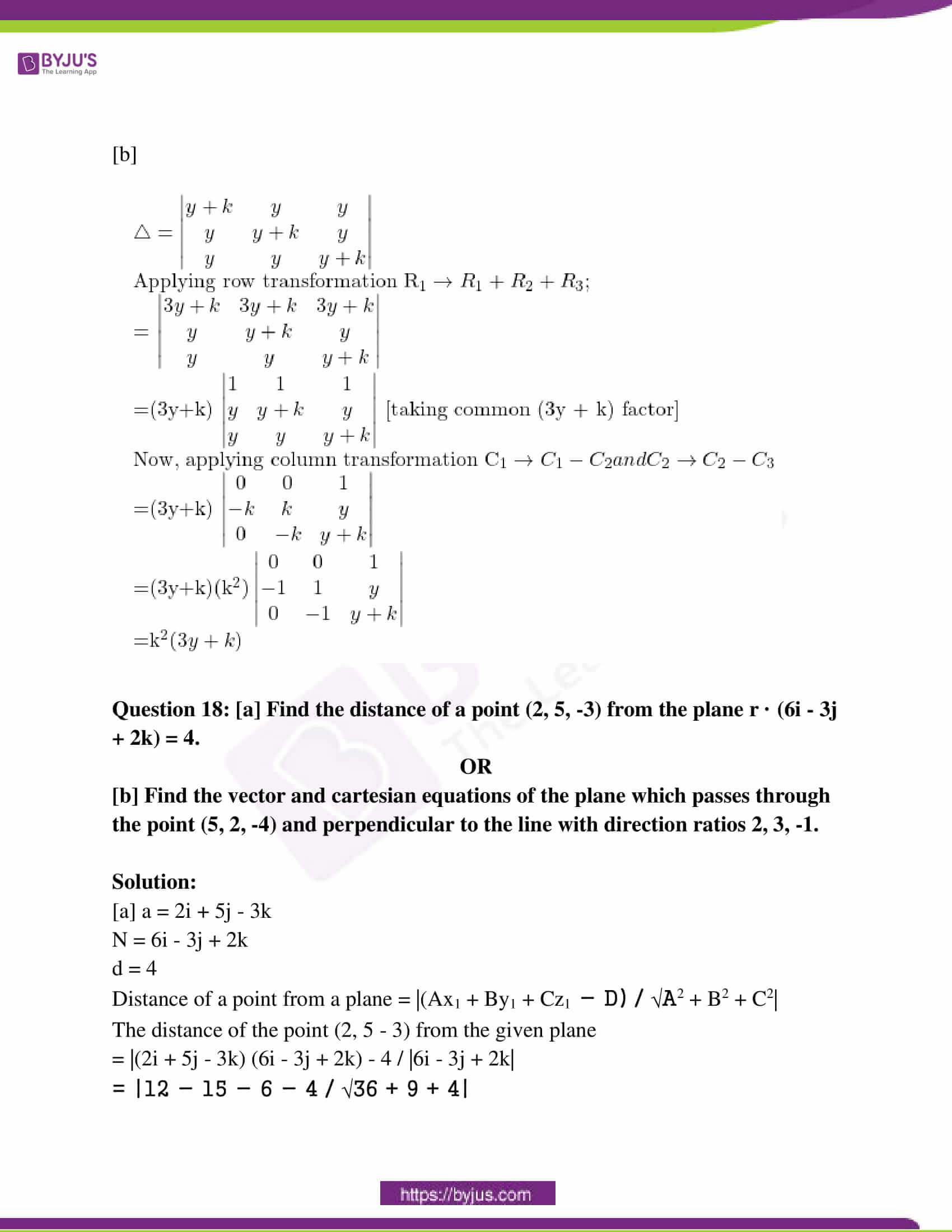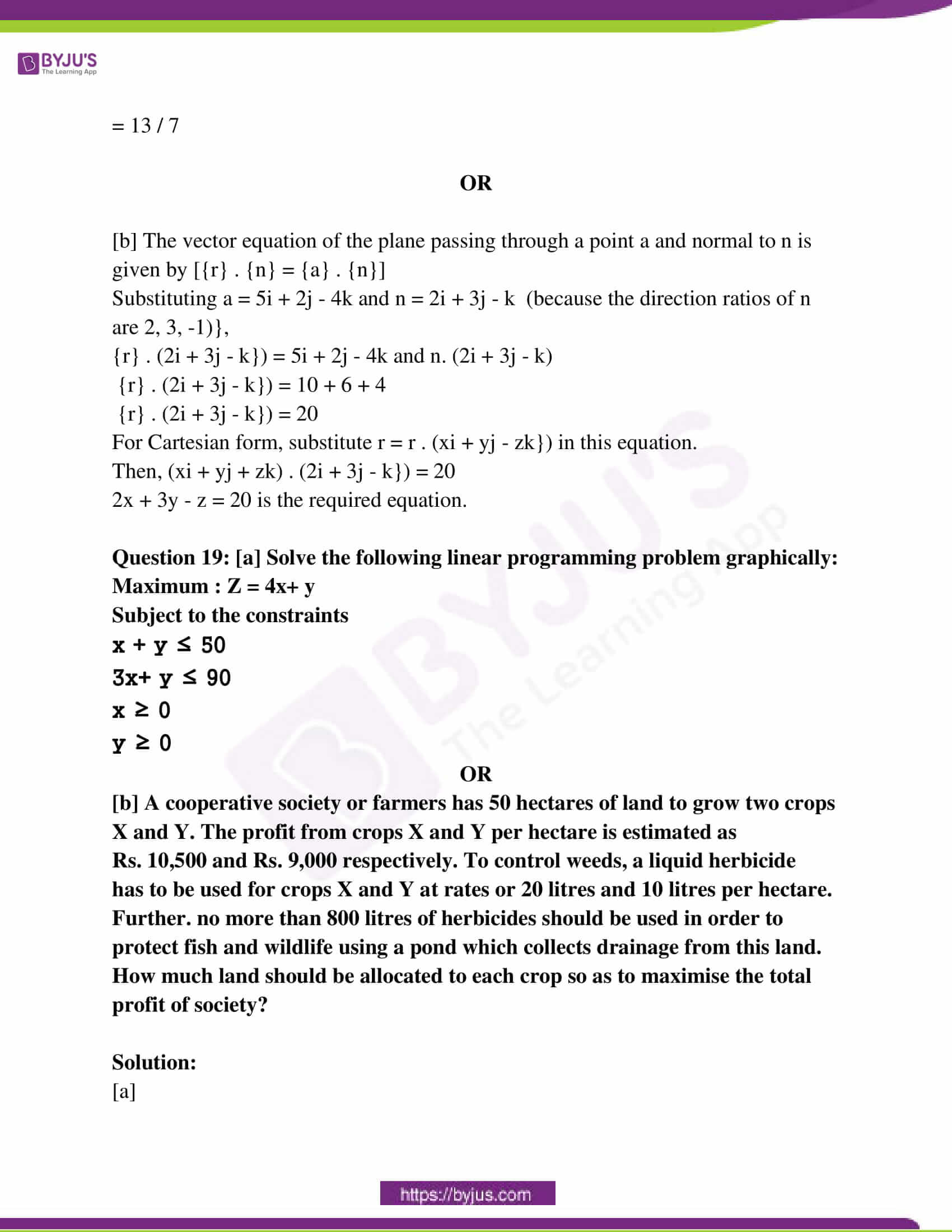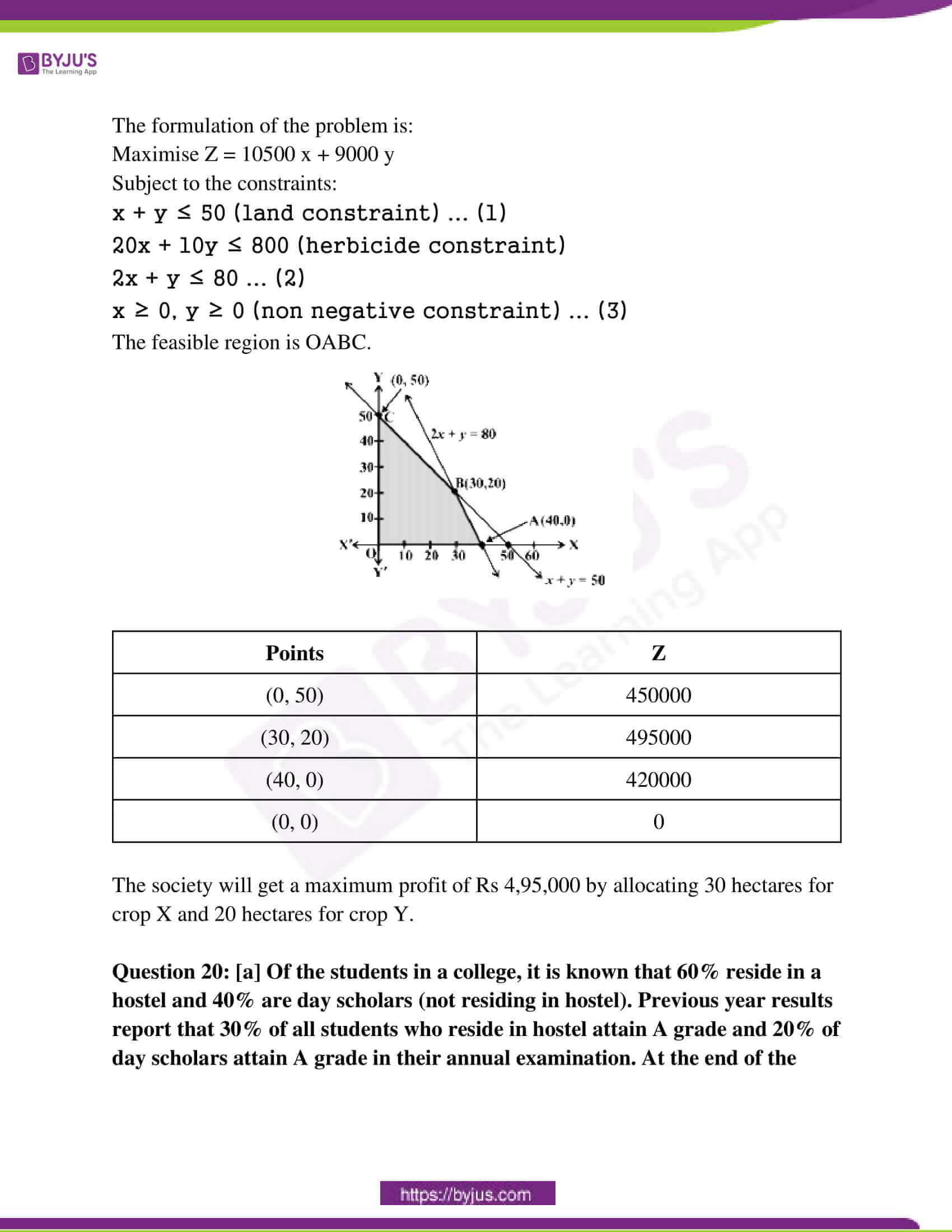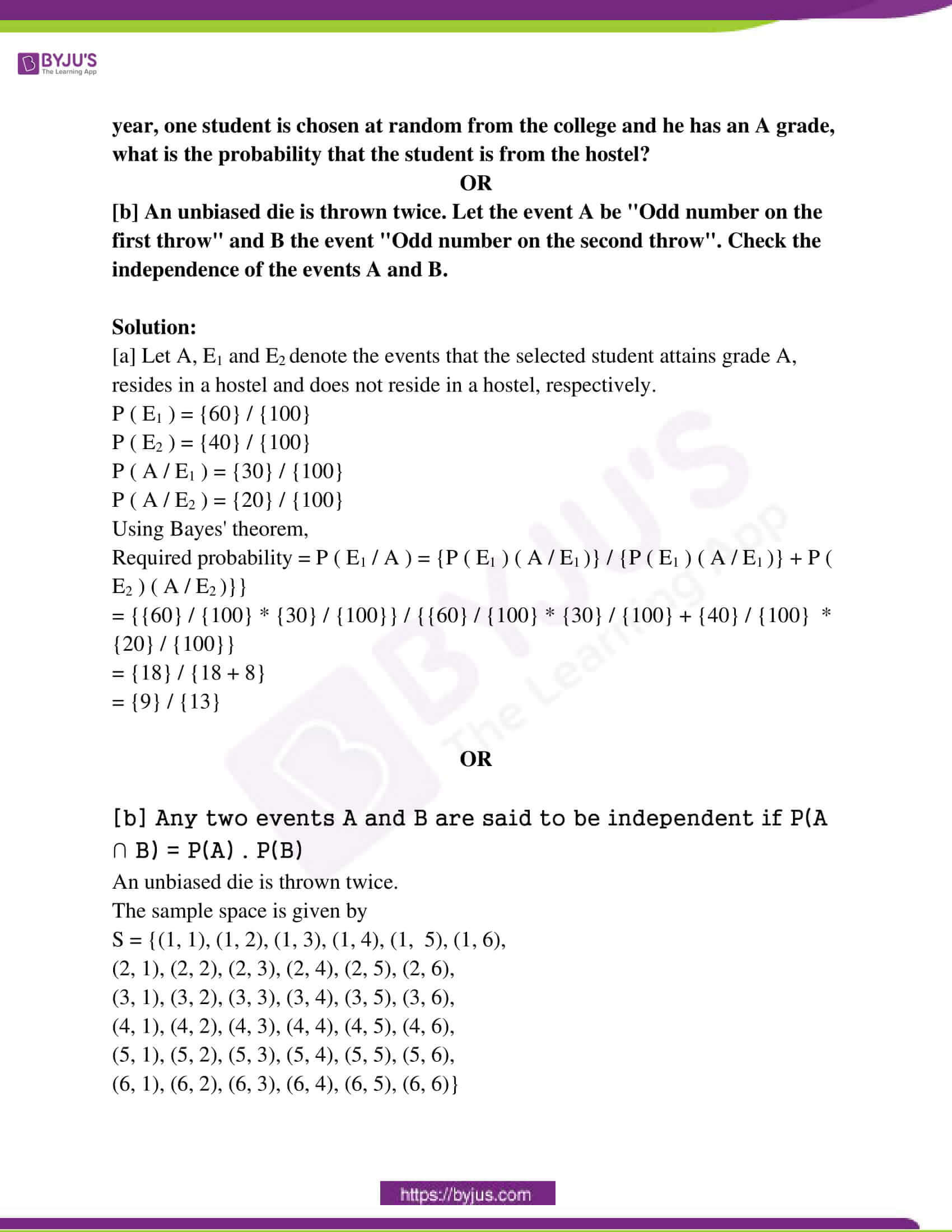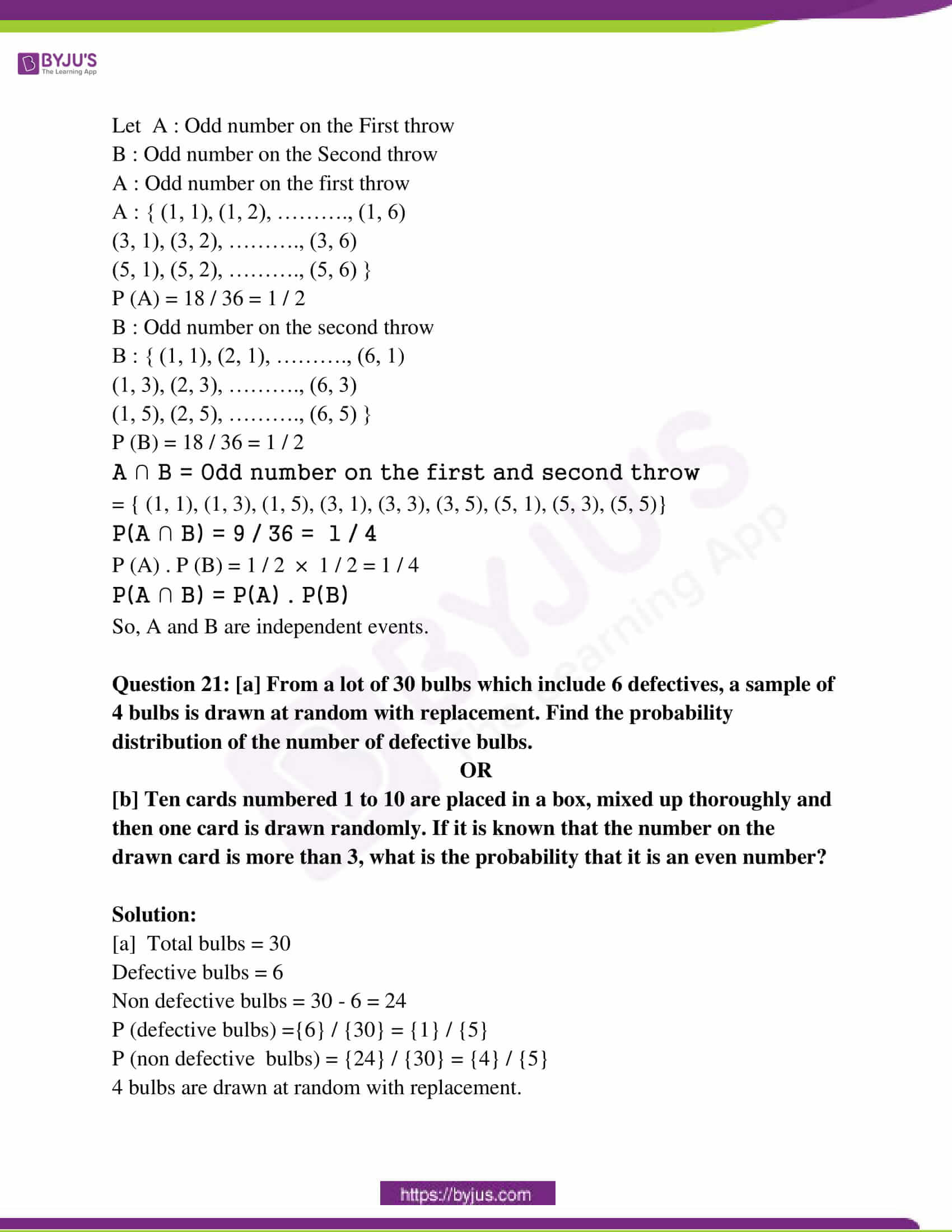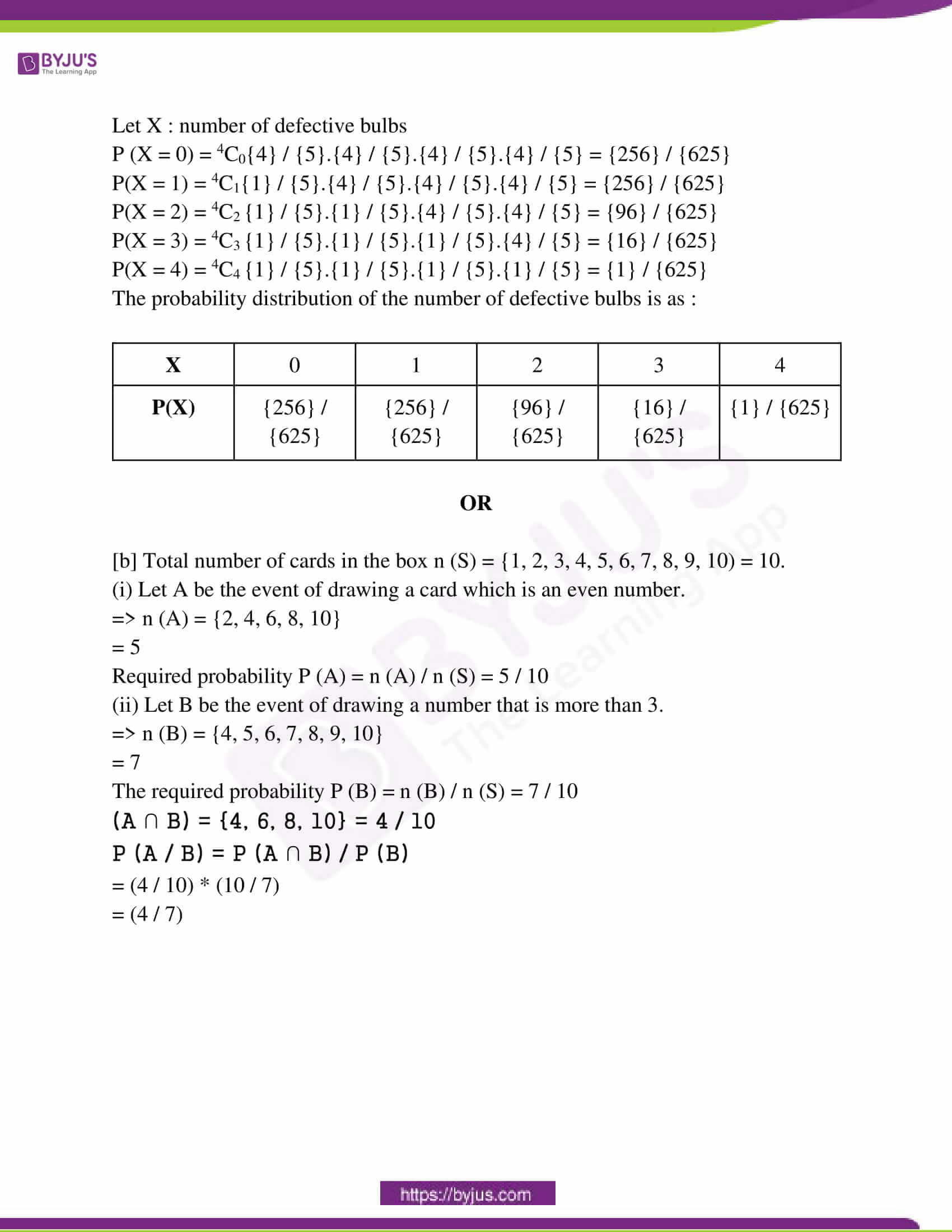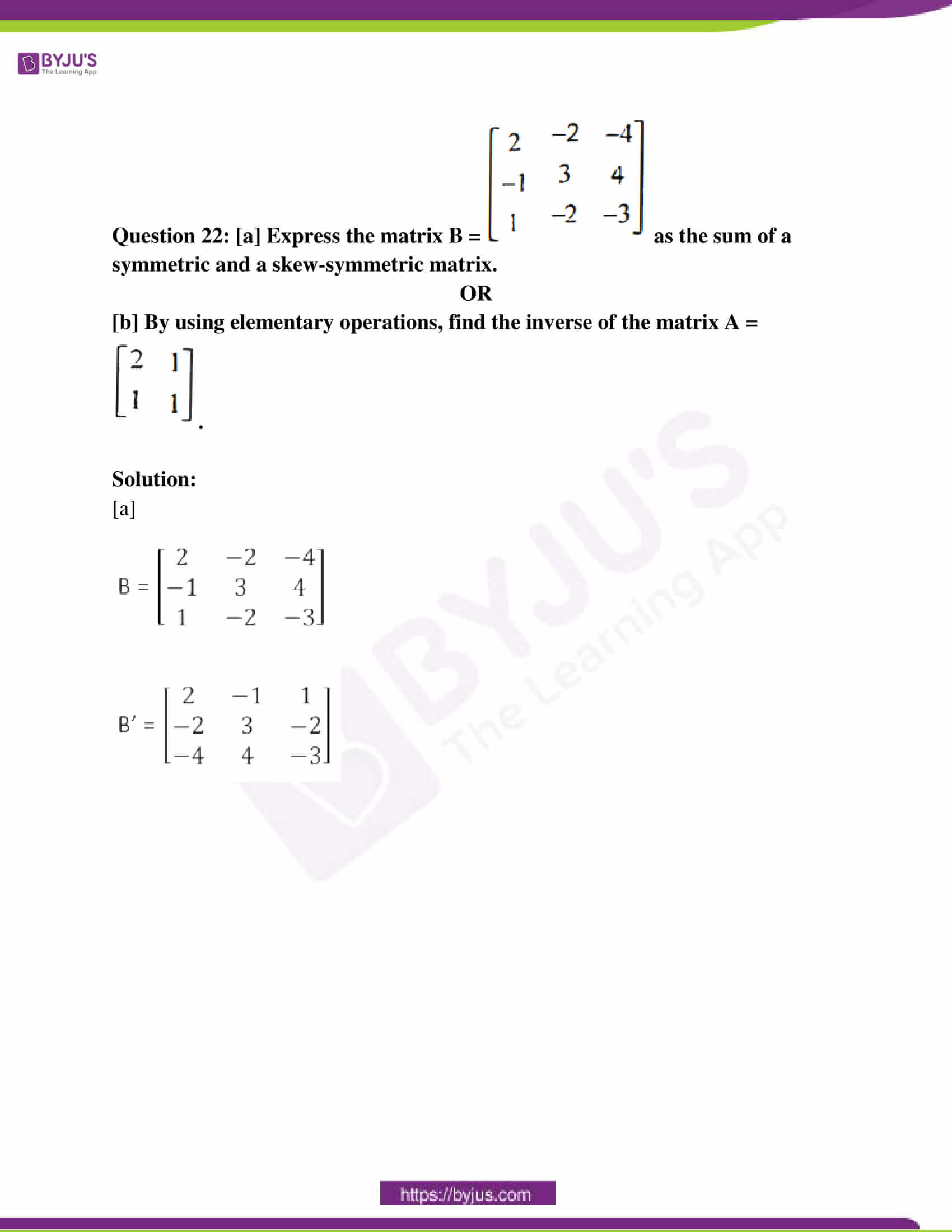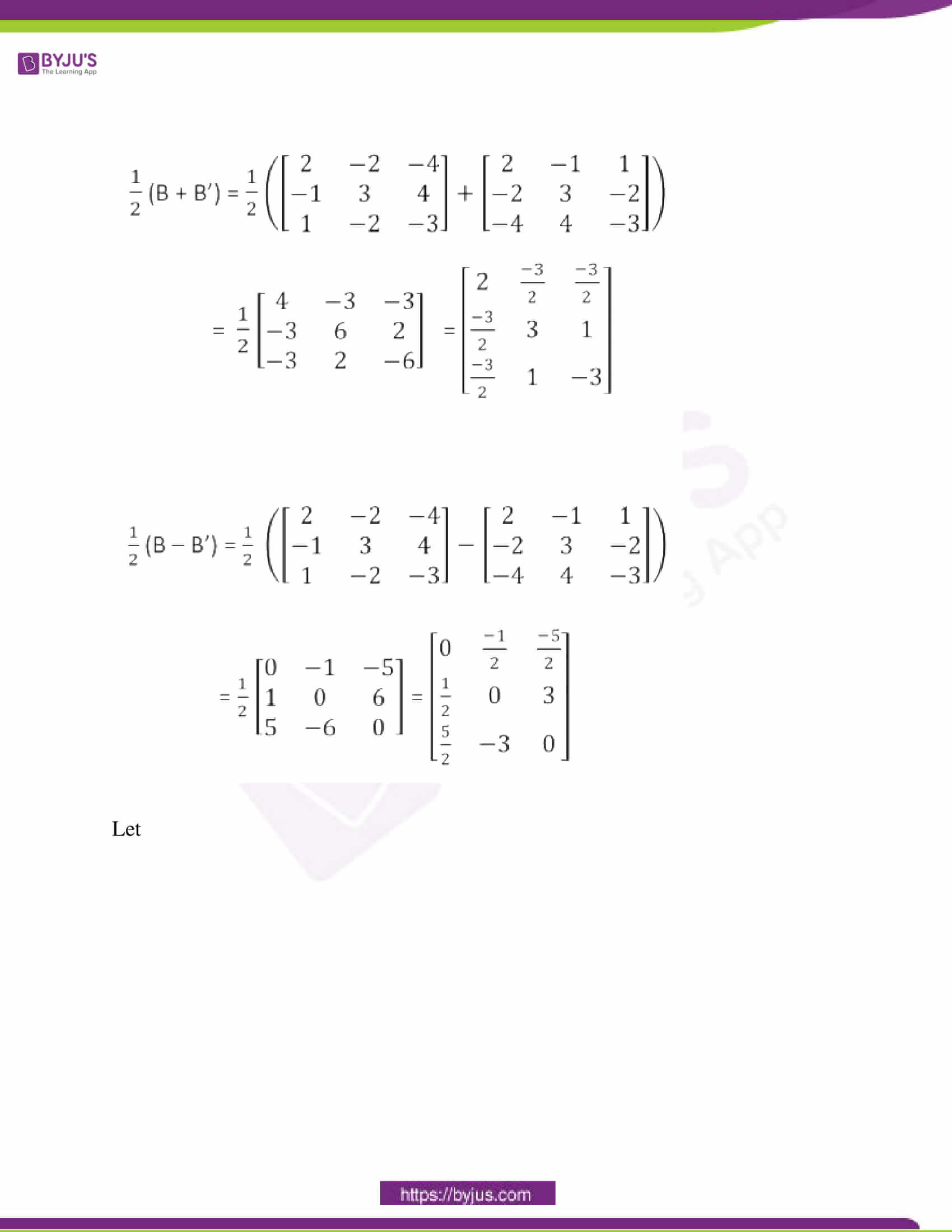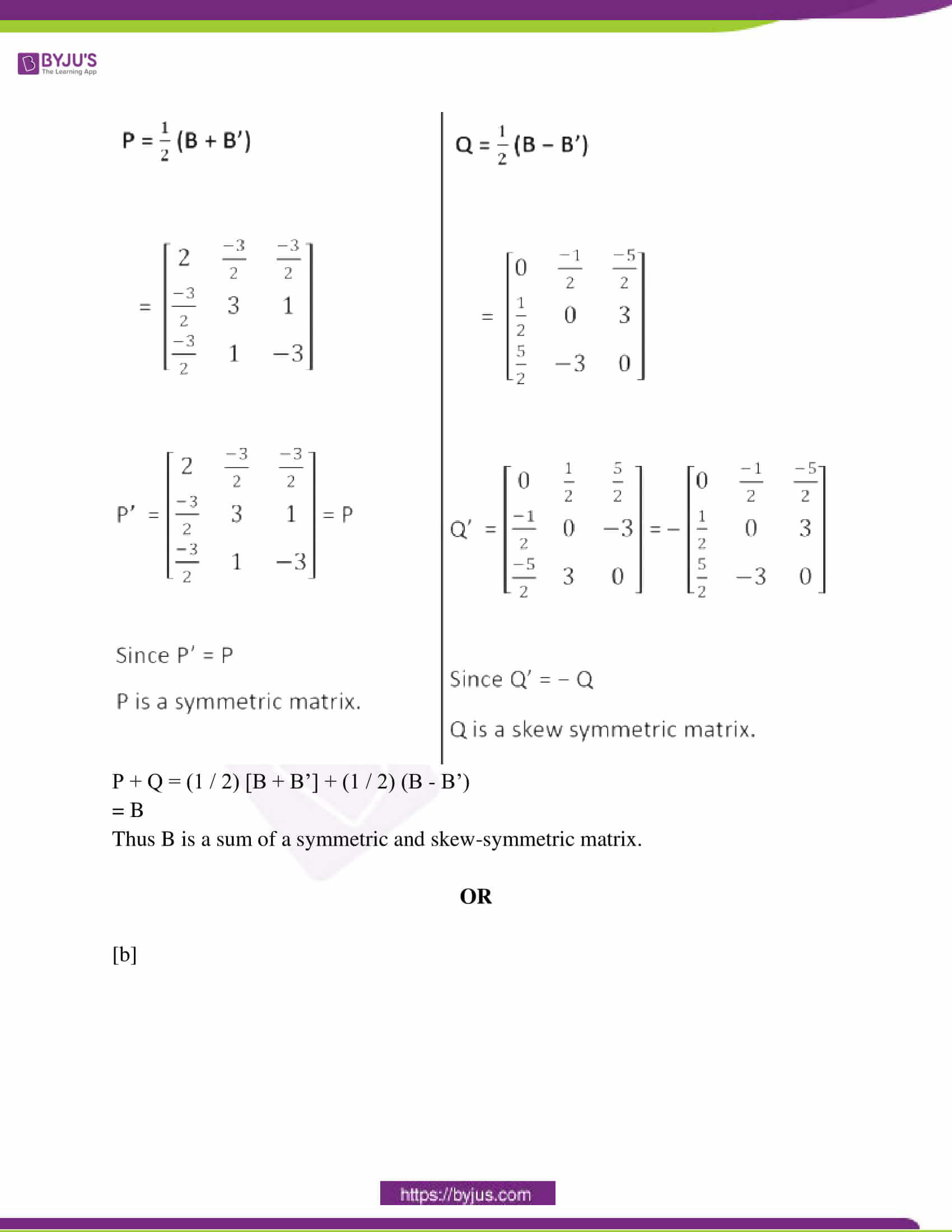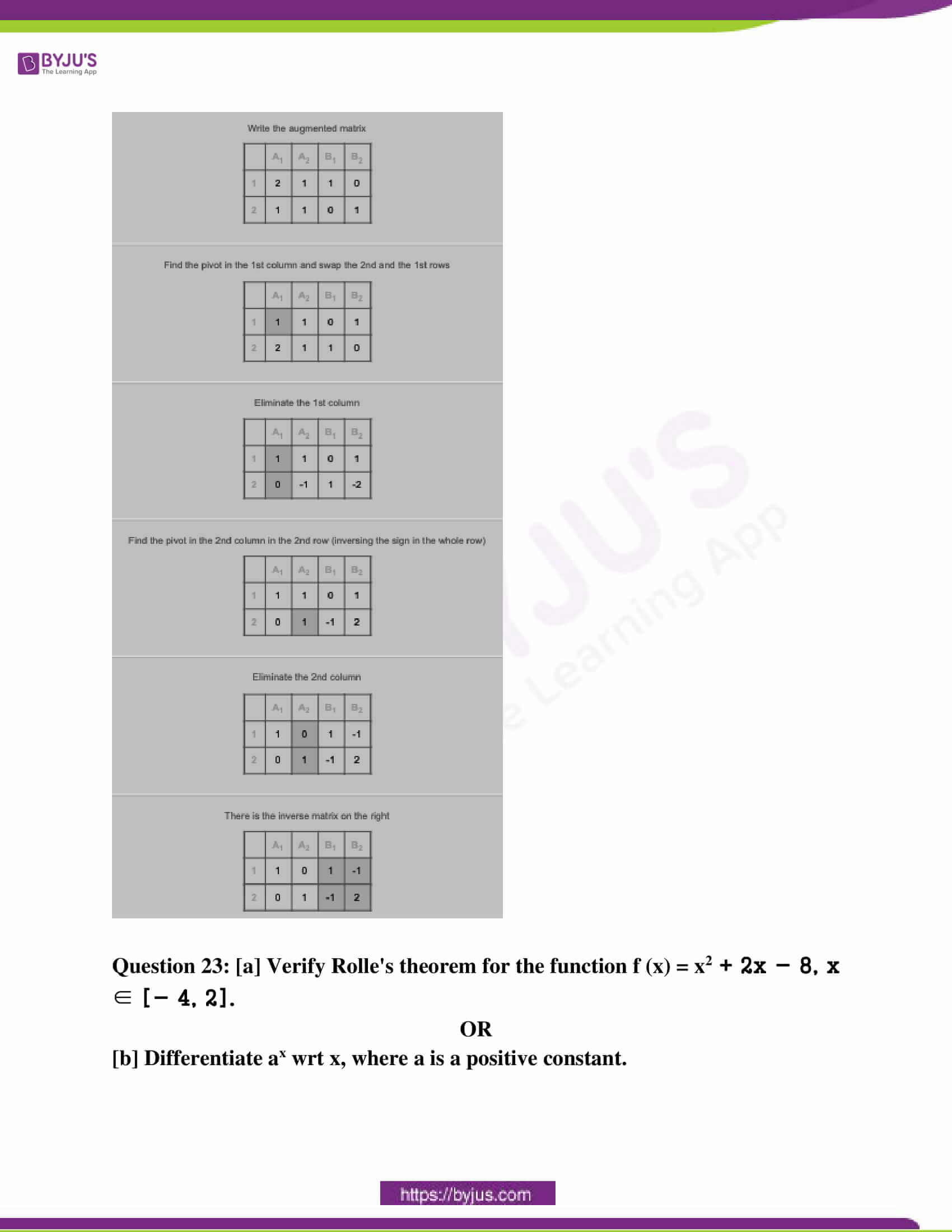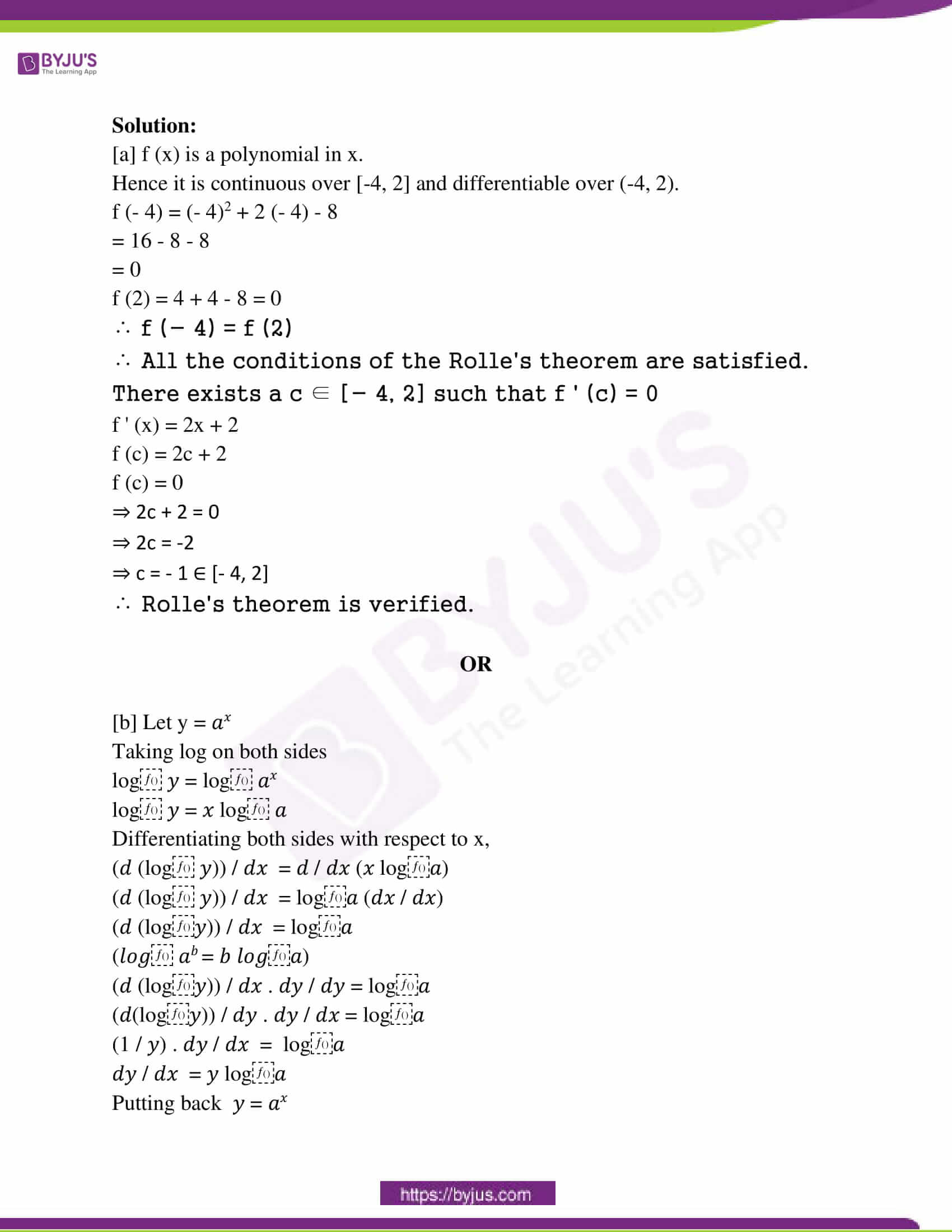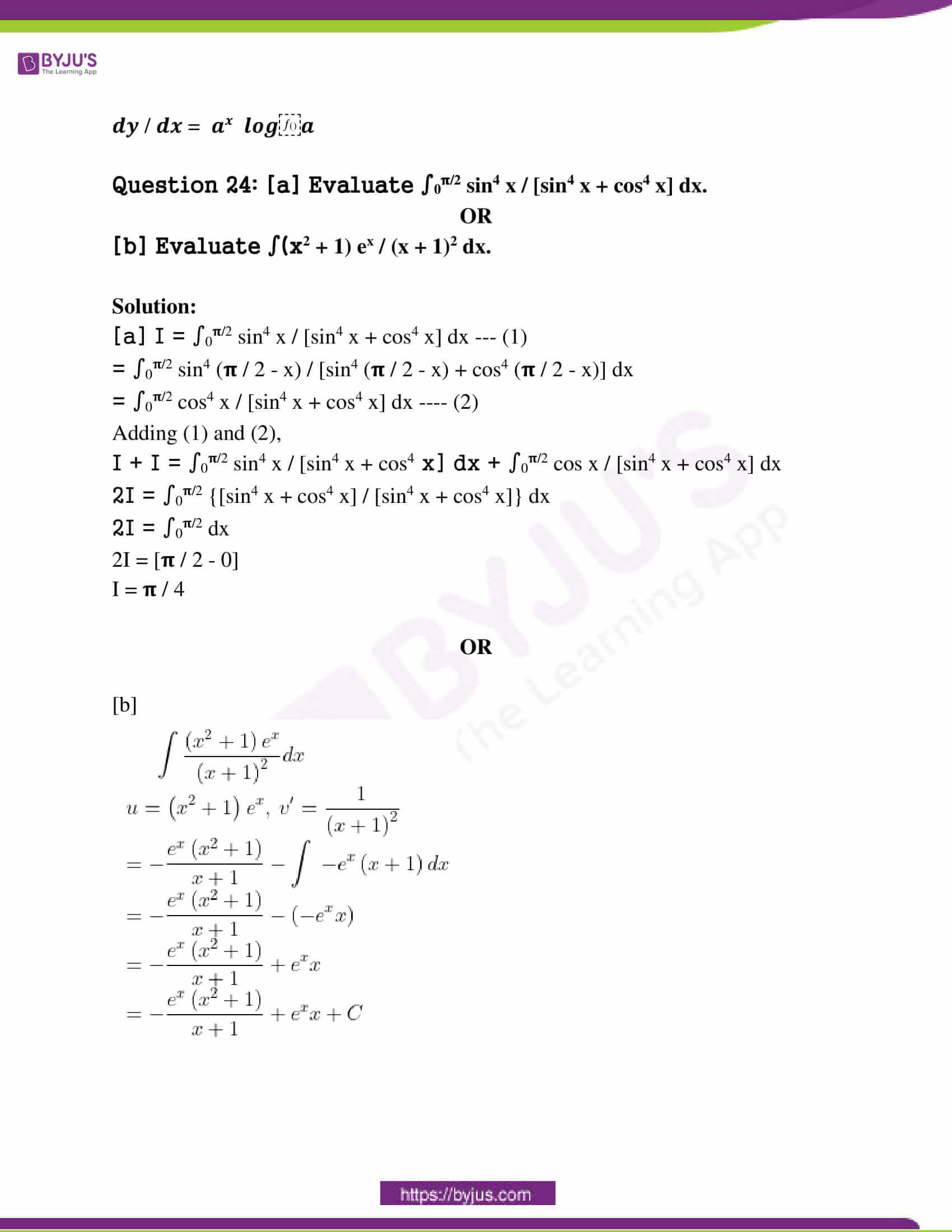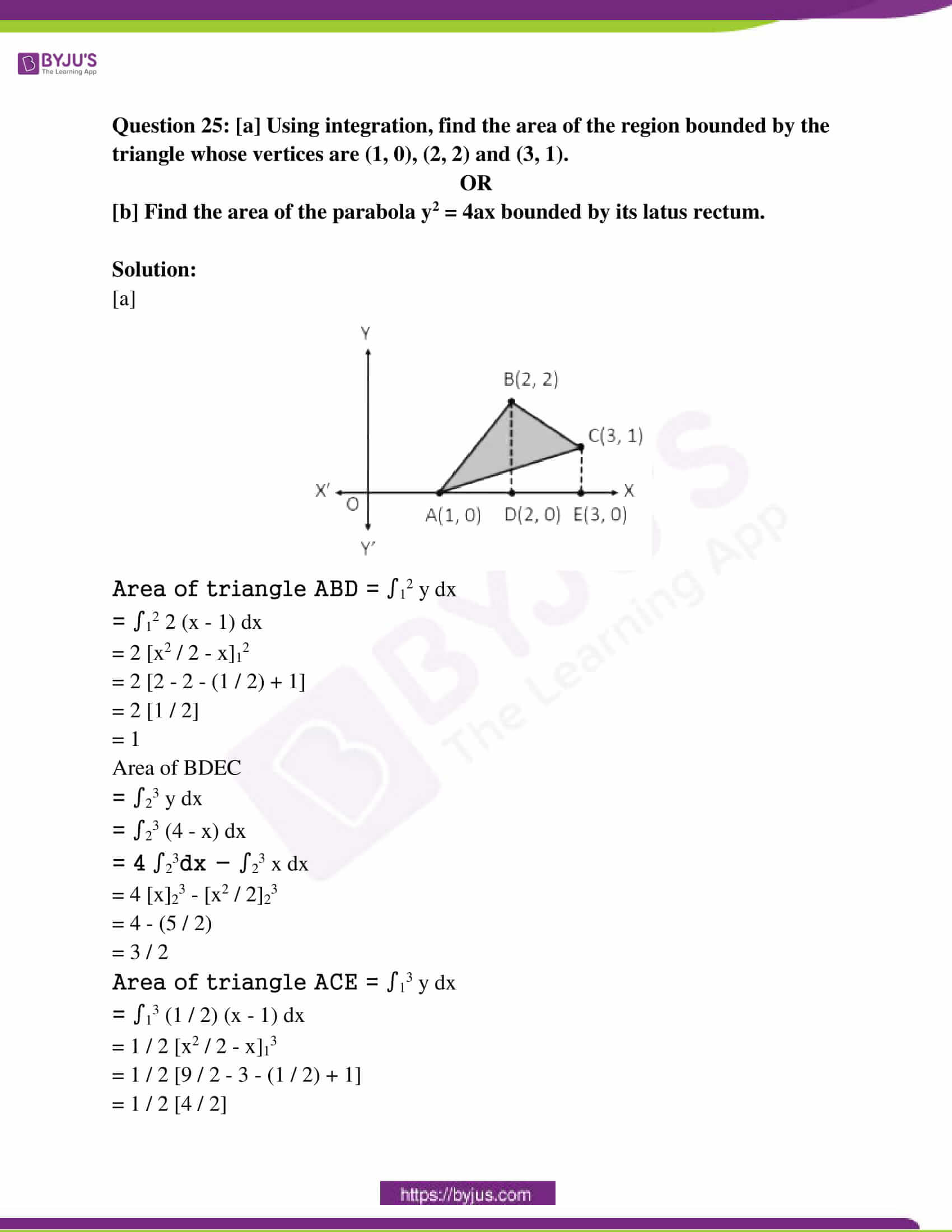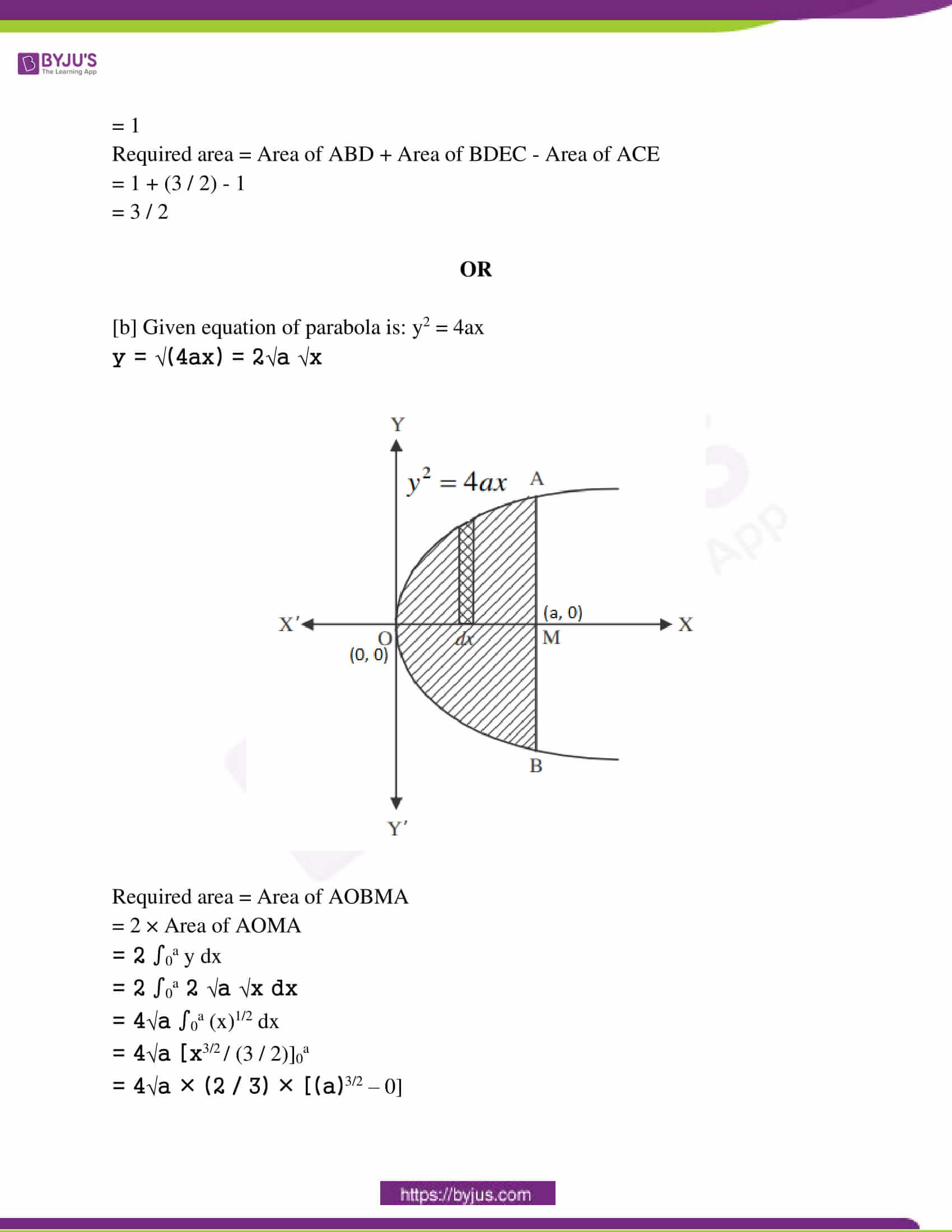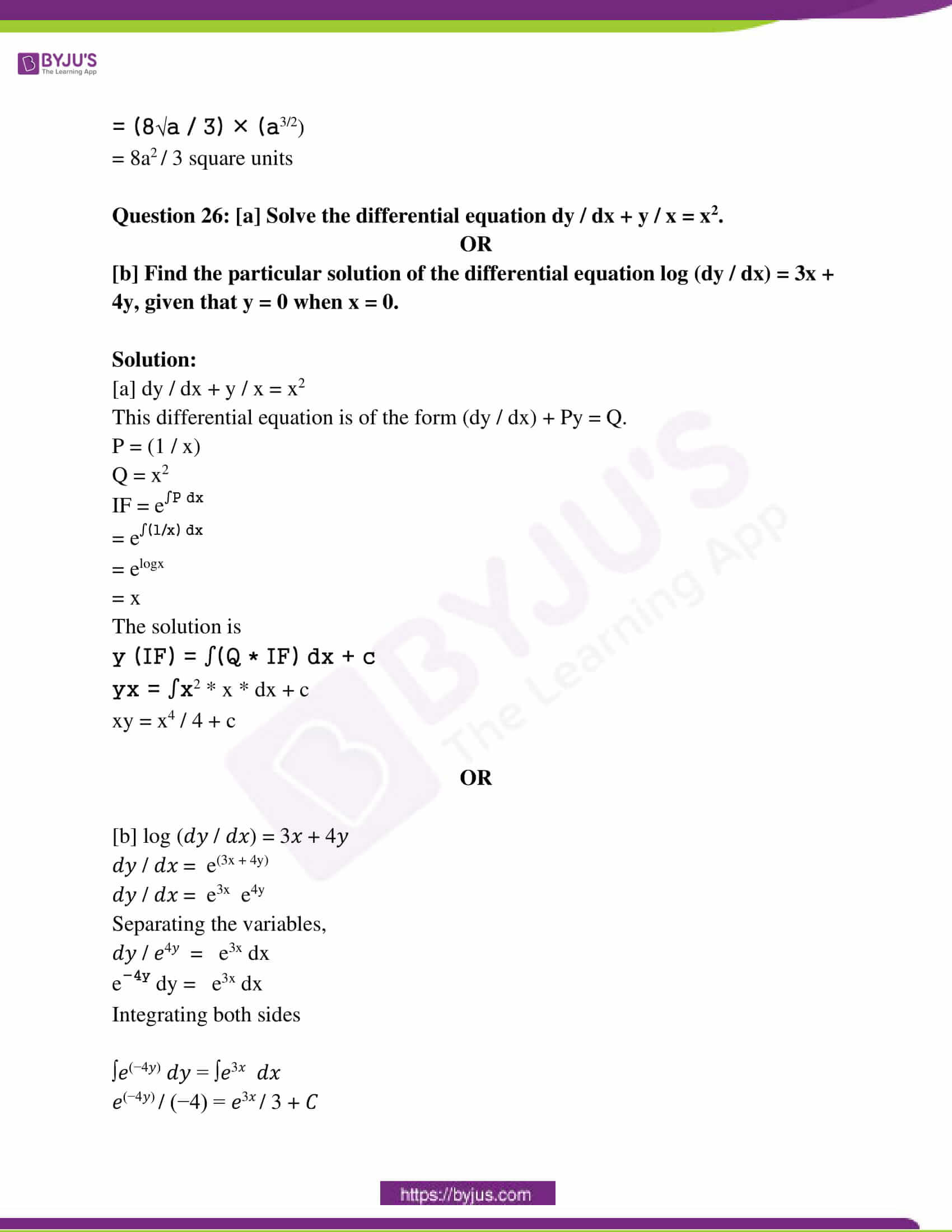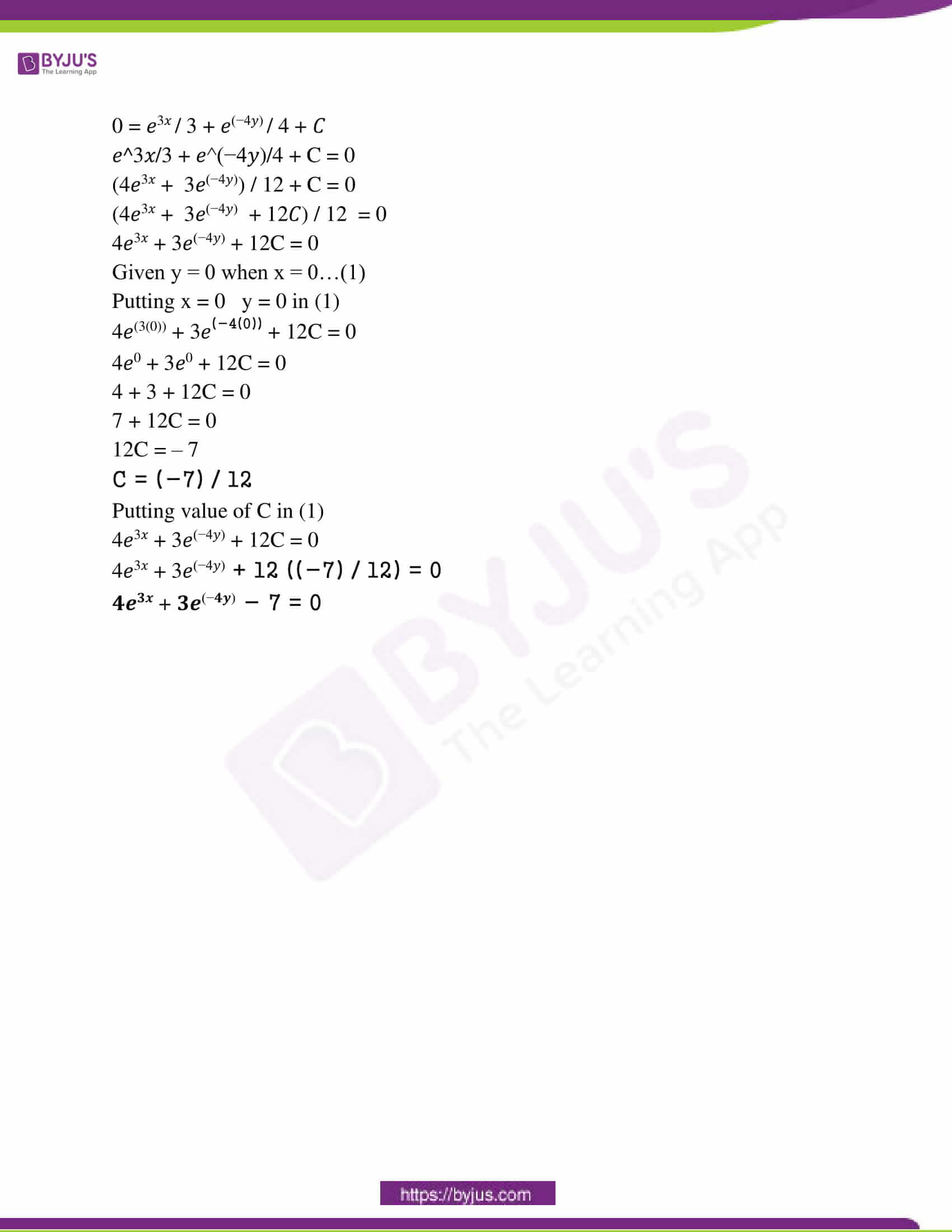SECTION – A

Question 1: Choose and write the correct options. 5M

[i] Let A = {1, 2, 3}, then the number of relations containing (1, 2) and (1, 3) which are reflexive and symmetric but not transitive is:

[a] 1 [b] 2 [c] 3 [d] 4

Solution: [a]

The given set is A = {1, 2, 3}.

The smallest relation containing (1, 2) and (1, 3) which are reflexive and symmetric but not transitive is given by:

R = {(1, 1), (2, 2), (3, 3), (1, 2), (1, 3), (2, 1), (3, 1)}

This is because relation R is reflexive as (1, 1), (2, 2), (3, 3) ∈ R.

The relation R is symmetric since (1, 2), (2, 1) ∈ R and (1, 3), (3, 1) ∈ R.

The relation R is not transitive as (3, 1), (1, 2) ∈ R, but (3, 2) ∉ R.

If the two pairs are added, (3, 2) and (2, 3) (or both) to relation R, then relation R will become transitive.

Hence, the total number of desired relations is one.

[ii] tan-1 √3 – sec-1 [-2] is equal to:

[a] 𝛑 [b] – 𝛑 / 3 [c] 𝛑 / 3 [d] 2𝛑 / 3

Solution: [b]

Let y = tan-1 (√3)

tan y = √3

tan y = tan (𝛑 / 3)

The principal value of tan-1 (√3) is 𝛑 / 3.

Let y = sec-1 (- 2)

sec y = – 2

sec y = sec (2𝛑 / 3)

The principal value of sec-1 (- 2) is 2𝛑 / 3.

tan-1 √3 – sec-1 [-2]

= (𝛑 / 3) – (2𝛑 / 3)

= – 𝛑 / 3

[iii] The number of possible matrices of order 3 x 3 with each entry 0 or 1 is:

[a] 27 [b] 18 [c] 81 [d] 512

Solution: [d]

Let A be a matrix of order 3 x 3.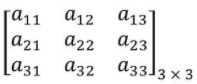There are a total of 9 elements.

Each item can be filled in 2 ways.

The number of matrices possible = 29 = 512

[iv] If A is an invertible matrix of order 2, then det (A-1) is equal to:

[a] det (A) [b] 1 / det (A) [c] 1 [d] 0

Solution: [b]

AA-1 = I

Taking determinant on both sides,

|A ||A-1| = 1

Divide by |A| both sides,

|A-1| = 1 / |A|

[v] If f (x) = ∫0x t sint dt, then f ‘(x) is

[a] cosx + x sinx [b] x sinx [c] x cosx [d] sinx + x cosx

Solution: [b]

f (x) = ∫0x t sint dt

Using integration by parts, t is the first function and sint is the second function.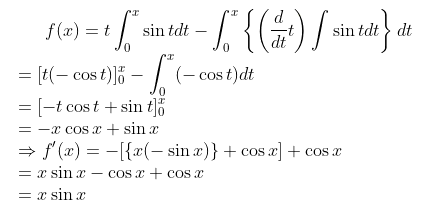Question 2: Fill in the blanks. 5M

[i] Every differentiable function is _____ [continuous].

[ii] Area lying in the first quadrant and bounded by the circle x2 + y2 = 4 and the lines x = 0 and y = 2 is _____. [𝛑]

[iii] The number of arbitrary constants in the general solution of a differential equation of fourth-order are _____. 

[iv] The length or the perpendicular from origin O to the plane r . N = d is _____. [d / |N|]

[v] The common region determined by all the constraints including the

non-negative constraints x ≥ 0, y ≥ 0 of a linear programming problem

is called _____ for the problem. [Feasible Region]

Question 3: Write true/false in the following statements. 5M

(i) If A and B Are two events such that P (A) ≠ 0 and P (B / A) = 1, then A ⊂ B. [True]

(ii) The probability that A speaks truth is 4 / 5. A coin is tossed. A reports that

a head appears. The probability that there was actually a head is 4 / 5. [True]

(iii) Any point outside the feasible region is the feasible solution. [False]

(iv) If 1, m, n are the direction cosines of a line, then l2 + m2 + n2 = 0. [False]

(v) The vector sum of the three sides of a triangle taken in order is 0. [True]

Question 4: Match the correct pairs. 5M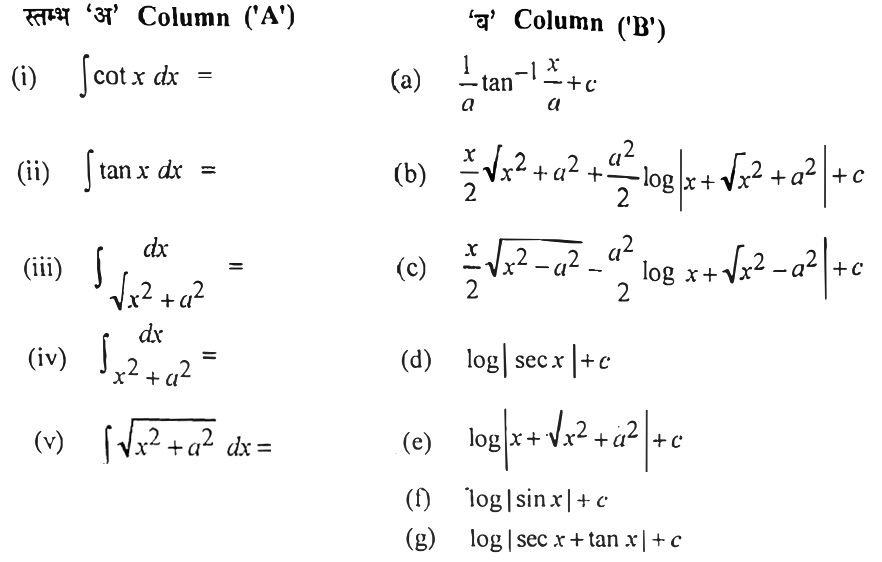Solution:

(i) (f)

(ii) (d)

(iii) (e)

(iv) (a)

(v) (b)

Question 5: Give answers in one word/sentence each. 5M

(i) What is the rate of change of the area of a circle with respect to its radius r at r = 6 cm?

Solution:

The area of a circle (A) with radius (r) is given by, A = πr2.

The rate of change of the area with respect to its radius r is

dA / dr = d (πr2) / dr = 2πr

∴When r = 6 cm,

dA dr = 2π × 6

= 12π cm2/s

The required rate of change of the area of a circle is 12π cm2/s.

(ii) Write the equation of the normal to the curve x2 = 4y which passes

through the point (1, 2).

Solution:

h2 = 4k

The slope of normal = −1 / [dy / dx] = −2h

The equation of normal (y − k) = − 2h (x − h)

k = 2 + 2 / h (1 − h)

h2 / 4 = 2 + (2 / h) (1 − h)

h = 2

k = 1

The equation of line is given by

(y – 1) = – 1 (x – 2)

x + y = 3

(iii) If f (x) = 3x2 + 15x + 5, then find the approximate value of f (3.02).

Solution:

Let x = 3, Δx = 0.02,

f (3.02) = f (x + Δx) = 3 (x + Δx)2 + 15 (x + Δx) + 5

Δy = f (x + Δx) – f (x)

f (x + Δx) = f (x) + Δy

= f (x) + f ‘ (x) Δx

f (3.02) = [3x2 + 15x + 5] + (6x + 15) Δx

= [3 (3)2 + 15 (3) + 5] + [6 (3) + 15] (0.02)

= (27 + 45 + 5) + (18 + 15) (0.02)

= 77 + (33) (0.02)

= 77 + 0.66

= 77.66

(iv) Write the slope of the normal to the curve y = 2x2 + 3 sin x at x = 0.

Solution:

The equation of the given curve y = 2x2 + 3 sin x.

The slope of the tangent to the given curve at x = 0 is given by,

(dy / dx)x=0 = (4x + 3 cosx)x=0

= 0 + 3 cos 0

= 3

Hence the slope of the normal to the given curve at x = 0 is

(- 1 / slope of the tangent at x = 0)

= – 1 / 3

(v) The total revenue in Rupees received from the sale of x units of a

product is given by R (x) = 3x2 + 36x + 5 . Write the marginal revenue,

when x = 15.

Solution:

R (x) = 3x² + 36x + 5

Marginal revenue = d R (x) / dx

= d (3x² + 36x + 5) / dx

= 6x + 36

When x = 15, marginal revenue = 6 (15) + 36

MR = 90 + 36

= 126

SECTION – B

Question 6: [a] Find X and Y if,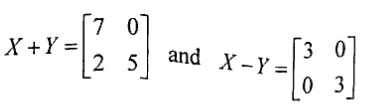.

OR

[b] Compute the indicated product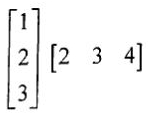.

Solution:

[a]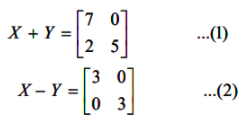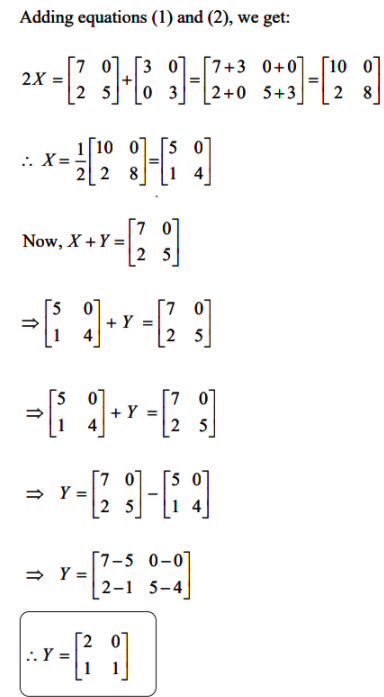OR

[b]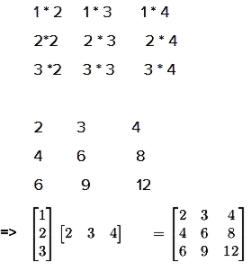Question 7: [a] Examine whether the function f given by f (x) = x2 is continuous at x = 0.

OR

[b] Find dy / dx, if x – y = 𝛑.

Solution:

[a] Given function is f (x) = x2.

It is continuous at x = 0 if lim x→0 f (x) = f (0).

LHS = lim x→0 f (x)

= lim x→1 x2

= 0

RHS = f (0)

= 0

lim x→0 f (x) = f (0)

Hence f is continuous at x = 0.

OR

[b] x – y = 𝛑

y = x – 𝛑

(dy / dx) = d (x – 𝛑) / dx

(dy / dx) = d (x) / dx

(dy / dx) = 1

Question 8: [a] Evaluate ∫{sin [tan-1 x] / (1 + x2)} dx.

OR

[b] Evaluate ∫(1 – x) √x dx.

Solution:

[a] ∫{sin [tan-1 x] / (1 + x2)} dx

Put tan-1 x = t

dt = dx / (1 + x2)

= ∫sin t dt

= – cost + c

= – cos (tan-1 x) + c

OR

[b]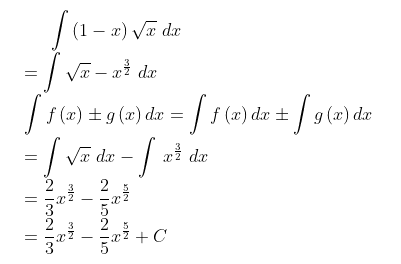Question 9: [a] Find the angle between two vectors a and b with magnitudes 1 and 2 respectively and when a . b = 1.

OR

[b] Find a vector in the direction of vector a = i – 2 j that has magnitude 7 units.

Solution:

[a] a . b = |a| |b| cos θ ; where θ is the angle between a and b

|a| = 1

|b| = 2

a . b = 1

Substitute the values in the above formula,

1 = 1 * 2 * cos θ

cos θ = 1 / 2

θ = cos-1 (1 / 2)

θ = 𝛑 / 3

OR

[b] Given vector is a = i – 2j = 1i – 2j + 0k

|a| = √12 + (-2)2 + 0

|a| = √5

Unit vector in direction of a = [1 / (magnitude of a)] * a

a = (1 / √5) (1i – 2j + 0k)

= (1 / √5) i – (2 / √5) j + 0k

= (1 / √5) i – (2 / √5) j

Vector having magnitude 1 = (1 / √5) i – (2 / √5) j

Vector having magnitude 7 = (7 / √5) i – (14 / √5) j

Question 10: [a] Find the direction cosines of x, y and z-axis.

OR

[b] Find the equation of the line which passes through the point (1, 2, 3) and is parallel to the vector 3i + 2j – 2k.

Solution:

[a] The x-axis makes an angle 0o with the x-axis, 90o with y-axis and 90o with the z-axis.

ɑ = 0o, β = 90o, 𝝲 = 90o

Direction cosines are

l = cos 0

m = cos 90

n = cos 90

l = 1

m = 0

n = 0

Direction cosines of x-axis are 1, 0, 0.

The y-axis makes an angle 90o with the x-axis, 0o with y-axis and 90o with the z-axis.

ɑ = 90o, β = 0o, 𝝲 = 90o

l = cos 90

m = cos 0

n = cos 90

l = 0

m = 1

n = 0

Direction cosines of the y-axis are 0, 1, 0.

The z-axis makes an angle 90o with the x-axis, 90o with y-axis and 0o with the z-axis.

ɑ = 90o, β = 90o, 𝝲 = 0o

l = cos 90

m = cos 90

n = cos 0

l = 0

m = 0

n = 1

Direction cosines of the y-axis are 0, 0, 1.

OR

[b] It is given that the line passes through the point A (1, 2, 3).

The position vector through A is a = i + 2j + 3k and b = 3i + 2j – 2k.

The line which passes through point A and parallel to b is given by

r = a + ƛb

r = i + 2j + 3k + ƛ (3i + 2j – 2k)

This is the required equation of the line.

Question 11: [a] A stone is dropped into a quiet lake and waves move in circles at a speed of 4 cm per second. At the instant, when the radius of the circular wave is 10 cm, how fast is the enclosed area increasing?

OR

[b] Use differential to approximate √36.6.

Solution:

[a] Consider r to be the radius of the circle and A be the area of the circle.

dr / dt = 4 cm/sec.

Area of circle = 𝛑r2

dA / dt = d (𝛑r2) / dt

= 𝛑 d (r2) / dt

= 𝛑 [(d (r2) / dt) * (dr / dr)]

= 𝛑 [2r * (dr / dt)]

= 𝛑 [2r * (4)]

= 𝛑 [8r]

When r = 10

(dA / dt)r=10 = 𝛑 * 8 * 10

= 80𝛑

dA / dt = 80𝛑 cm2/sec

OR

[b] Let y = √x

Let x = 36 & Δx = 0.6

y = √x

dy / dx = d (√x) / dx

= 1 / 2 (√x)

Δy = [dy / dx] Δx

= (1 / 2) (√x) (0.6)

= (1 / 2) √36 (0.6)

= 0.05

Δy = f (x + Δx) – f (x)

0.05 = √36 + 0.6 – √36

√36.6 = 0.05 + √36

√36.6 = 0.05 + 6

Δy = √x + Δx – √x

√36.6 = 6.05

Hence the approximate value of √36.6 is 6.05.

Question 12: [a] Find two positive numbers whose sum is 15 and the sum of whose squares are minimum.

OR

[b] If the radius of a sphere is measured as 9 cm with an error 0.03 cm, then find the approximate error in calculating its volume.

Solution:

[a] Let the first number is x.

It is given that the sum of two numbers is 15.

⇒ 1st number + 2nd number = 15

⇒ x + 2nd number = 15

⇒ 2nd number = 15 – x

⇒ f (x) = x² + (15 – x)² = 2x² – 30x + 225

f ‘ (x) = 4x – 30

For maximum or minimum, f ‘ (x) = 0,

⇒ 4x – 30 = 0

⇒ x = 7.5

Again differentiating f ‘ (x) with respect to x,

f ” (x) = 4

At x = 7.5 f ” (x) = +ve,

f (x) is minimum at x = 7.5

The first number is 7.5 and the second number is 15 – 7.5 = 7.5.

OR

[b] Let r be the radius of the sphere, r = 9 cm

Error in measurement of radius= Δr = 0.03 cm

Volume of the sphere = V = (4 / 3) 𝛑r3

ΔV = (dv / dr) Δr

= d ((4 / 3) 𝛑r3) / dr) Δr

= (4 / 3) 𝛑 (dr3 / dr) Δr

= (4 / 3) 𝛑 (3r2) (0.03)

= 4 (9)2 (0.03)

= 9.72𝛑cm3

The error in calculating the volume is 9.72 cm3.

Question 13: [a] Find a unit vector perpendicular to each of the vectors (a + b) and (a – b ), where a = i + j + k , b = i + 2j + 3k.

OR

[b] Show that the point A (-2i + 3j + 5k), B (i + 2j + 3k) and C (7i – k) are collinear.

Solution:

[a] 𝑎 = 𝑖 + 𝑗 + 𝑘= 1𝑖 + 1𝑗 + 1𝑘

𝑏 = 𝑖 + 2𝑗 + 3𝑘 = 1𝑖 + 2𝑗 + 3𝑘

(a + b) = (1 + 1) 𝑖 + (1 + 2) 𝑗 + (1 + 3) 𝑘

= 2𝑖 + 3𝑗 + 4𝑘

(a – b) = (1 − 1) 𝑖 + (1 − 2) 𝑗 + (1 − 3) 𝑘

= 0𝑖 − 1𝑗 − 2𝑘

Let (a + b) × (a – b) = c

𝑐 =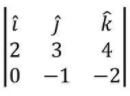= 𝑖 [(3 × −2) − (−1 × 4)] −𝑗 [(2 × −2) − (0 × 4)] + 𝑘 [(2 × −1) − (0 × 3)]

= 𝑖 [−6 − (−4)] −𝑗 [−4 −0] + 𝑘 [−2 −0]

= 𝑖 (−6 + 4) −𝑗 (−4) + 𝑘 (−2)

= −2𝑖 + 4𝑗 − 2𝑘

∴ 𝒄 = -2𝒊 + 4𝒋 − 2𝒌

The unit vector of 𝑐 = [(1 / (magnitude of 𝑐 ) × 𝑐]

|c| = √((−2)2 + (4)2 + (−2)2)

|𝑐 | = √(4 + 16 + 4)

= √24

= √(2 × 2 × 6)

= 2√6

Unit vector of 𝑐 = (1 / |𝑐|) × 𝑐

= (1 / (2√6)) [−2𝑖 + 4𝑗 − 2𝑘]

= (1 / (2√6)) × 2 [−𝑖 + 2𝑗 − 𝑘]

= (−1) / √6 𝑖 + 2 / √6 𝑗 − 1 / √6 𝑘

The required unit vector is = (−1) / √6 𝑖 + 2 / √6 𝑗 − 1 / √6 𝑘.

OR

[b] AB = (1 + 2)i + (2 – 3)j + (3 – 5)k = 3i – j – 2k

BC = (7 – 1)i + (0 – 2)j + (-1 – 3)k = 6i – 2j – 4k

CA = (7 + 2)i + (0 – 3)j + (-1 – 5)k = 9i – 3j – 6k

|AB|2 = 14

|BC|2 = 56

|CA|2 = 126

|AB| = √14

|BC| = 2√14

|CA| = 3√14

⇒ |CA| = |AB| + |BC|

Hence the points A, B and C are collinear.

Question 14: [a] Find the angle between the lines (x + 1) / 2 = y / 3 = (z – 3) / 6 and the plane 10x + 2y – 11z = 3.

OR

[b] Show that lines (x + 3) / -3 = (y – 1) / 1 = (z – 5) / 5 and (x + 1) / -1 = (y – 2) / 2 = (z – 5) / 5 are coplanar.

Solution:

[a] The angle between a line (x − 𝑥1) / 𝑎 = (𝑦 − 𝑦1) / 𝑏 = (𝑧 − 𝑧1) / 𝑐 and the normal to the plane Ax + By + Cz = D is given by

cos θ = |(𝐴𝑎 + 𝐵𝑏 + 𝐶𝑐) / (√(𝑎2 + 𝑏2 + 𝑐2 ) √(𝐴2 + 𝐵2 + 𝐶2))|

The angle between line and plane is given by

sin ϕ = |(𝐴𝑎 + 𝐵𝑏 + 𝐶𝑐) / (√(𝑎2 + 𝑏2 + 𝑐2 ) √(𝐴2 + 𝐵2 + 𝐶2))|

The line is

(𝑥 + 1) / 2 = 𝑦 / 3 = (𝑧 − 3) / 6

(𝑥 − (−1)) / 2 = (𝑦 − 0) / 3 = (𝑧 − 3) / 6

Comparing with

(x − 𝑥1) / 𝑎 = (𝑦 − 𝑦1) / 𝑏 = (𝑧 − 𝑧1) / 𝑐

𝑎 = 2, b = 3, c = 6

The plane is 10x + 2y − 11z = 3.

On comparing with Ax + By + Cz = D,

A = 10, B = 2, C = −11

sin ϕ = |((10 × 2) + (2 × 3) + (−11 × 6)) / (√(22 + 32 + 62 ) √(102 + 22 + (−11)2))|

= |(20 + 6 − 66) / (√(4 + 9 + 36) √(100 + 4 + 121))|

= |(−40) / (7 × 15)|

= 8 / 21

sin ϕ = 8 / 21

ϕ = sin-1 (8 / 21)

OR

[b] The two lines (x − 𝑥1) / 𝑎1 = (𝑦 − 𝑦1) / 𝑏1 = (𝑧 − 𝑧1) / 𝑐1 and (x − 𝑥2) / 𝑎2 = (𝑦 − 𝑦2) / 𝑏2 = (𝑧 − 𝑧2) / 𝑐2 are coplanar if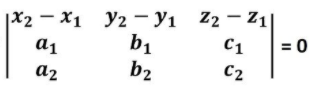(𝒙 + 𝟑) / ( − 𝟑) = (𝒚 − 𝟏) / 𝟏 = (𝒛 − 𝟓) / 𝟓

(𝑥 −(−3)) / (− 3) = (𝑦 − 1) / 1 = (𝑧 − 5) / 5

Comparing (x − 𝑥1) / 𝑎 = (𝑦 − 𝑦1) / 𝑏 = (𝑧 − 𝑧1) / 𝑐1

𝑥1 = −3, 𝑦1 = 1, 𝑧1= 5

a1 = −3, 𝑏1 = 1, 𝑐1= 5

(𝒙 + 𝟏)/( − 𝟏) = (𝒚 − 𝟐)/𝟏 = (𝒛 − 𝟓)/𝟓

(𝑥 − (−1))/( − 1) = (𝑦 − 2)/2 = (𝑧 − 5)/5

Comparing (x − 𝑥2) / 𝑎2 = (𝑦 − 𝑦2) / 𝑏2 = (𝑧 − 𝑧2) / 𝑐2

𝑥2 = −1, 𝑦2 = 2, 𝑧2= 5

𝑎2 = −1, 𝑏2 = 2, 𝑐2 = 5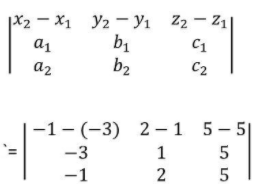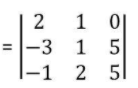= 2 [(1 × 5) − (2 × 5)] − 1[(−3 × 5) − (− 1 × 5)] + 0 [(−3 × 2) − (−1 × 1)]

= 2 [5 − 10] − 1 [− 15 − (− 5)] + 0

= 2 (−5) − 1 (−10)

= −10 + 10

= 0

Therefore, the given two lines are coplanar.

Question 15: [a] Check whether the relation R defined in the set {1, 2, 3, 4, 5, 6} as R = {(a, b): b = a + 1} is reflexive, symmetric or transitive.

OR

[b] Show that *: R x R ➔ R defined by a* b = a + 2b is neither commutative nor associative.

Solution:

[a] R = {(a, b): b = a + 1}

Reflexivity:

Let a be an arbitrary element of R.

Then, a = a + 1 cannot be true for all a ∈ A.

⇒ (a, a) ∉ R

So, R is not reflexive on A.

Symmetry:

Let (a, b) ∈ R

⇒ b = a + 1

⇒ −a = −b + 1

⇒ a = b − 1

Thus, (b, a) ∉ R

So, R is not symmetric on A.

Transitivity:

Let (1, 2) and (2, 3) ∈ R

⇒ 2 = 1 + 1 and 3

2 + 1 is true.

But 3 ≠ 1+1

⇒ (1, 3) ∉ R

So, R is not transitive on A.

OR

[b] * is associative if

(a * b) * c = a * (b * c)

(a * b) * c

= (a + 2b) * c

= (a + 2b) + 2c

= a + 2b + 2c

a * (b * c)

= a * (b + 2c)

= a + 2 (b + 2c)

= a + 2b + 4c

Since (a * b) * c ≠ a * (b * c), * is not an associative binary operation.

Question 16: [a] Express tan-1 (cosx / [1 – sinx]), – 3𝛑 / 2 < x < 𝛑 / 2 in the simplest form.

OR

[b] Show that sin-1 (3 / 5) – sin-1 (8 / 17) = cos-1 (84 / 85).

Solution:

[a] cos 2x = cos2 x – sin2 x

Replacing x by x / 2

cos (2x / 2) = cos2 x / 2 – sin2 x / 2

cos x = cos2 x / 2 – sin2 x / 2

sin 2x = 2 sin x cos x

Replacing x by 𝑥 / 2

sin (2𝑥 / 2) = 2 sin 𝑥 / 2 cos 𝑥 / 2

sin x = 2 sin 𝑥 / 2 cos 𝑥 / 2

tan−1 (cos⁡x / (1 − sin⁡x ))

= tan−1 [(cos2 x / 2 − sin2 x / 2) / (1 − (2 sin ⁡x / 2 cos⁡ x / 2))]

= tan−1 [(cos2 x / 2 − sin2 x / 2) / (1 − 2 sin x / 2 cos x / 2)]

= tan−1 [(cos2 x / 2 − sin2 x / 2) / (cos2 x / 2 + sin2 x / 2 − 2 sin ⁡x / 2 cos⁡ x / 2)]

As sin2 x + cos2 x = 1

Replacing x by 𝑥 / 2

sin2 𝑥 / 2 + cos2 𝑥 / 2 = 1

1 = sin2 𝑥 / 2 + cos2 𝑥/2

tan−1 (cos⁡x / (1 − sin⁡x )) = tan−1 [(cos2 x / 2 − sin2 x / 2) / (1 − (2 sin x / 2 cos⁡ x / 2))]

= tan−1 [(cos2 x / 2 − sin2 x / 2) / (1 − 2 sin x / 2 cos ⁡x / 2)]

= tan−1 [(cos2 x / 2 − sin2 x / 2) / (cos2 x / 2 + sin2 x / 2 − 2 sin x / 2 cos⁡ x / 2)]

= tan−1 [(cos x / 2 + sin x / 2) (cos x / 2 − sin x / 2) / (cos x / 2 − sin x / 2)2]

= tan−1 [(cos x / 2 + sin x / 2) (cos x / 2 − sin x / 2) / (cos x / 2 − sin x / 2)2 ]

= tan−1 [((cos x / 2 + sin x / 2)) / ((cos x / 2 − sin x / 2))]

Dividing by cos x / 2

= tan−1 ((cos⁡ x / 2 / cosx / 2 + sin⁡ x / 2 / cos ⁡x / 2) / (cos⁡ x / 2 / cos ⁡x / 2 − sin⁡ x / 2 / cos ⁡x / 2))

= tan−1 [(1 + tan ⁡x / 2) / (1 − tan⁡ x / 2)]

= tan−1 [(𝟏 + tan ⁡x / 2) / (1 − 𝟏 .tan⁡ x / 2)]

= tan−1 ((tan⁡ 𝝅 / 𝟒 + tan ⁡𝑥 / 2) / ( 1− tan ⁡𝝅 / 𝟒 . tan ⁡𝑥 / 2))

= tan−1 [tan ⁡(π / 4 + x / 2)]

= 𝛑 / 𝟒 + 𝐱 / 𝟐

OR

[b] sin-1 (3 / 5) = x and sin-1 (8 / 17) = y

sinx = 3 / 5

siny = 8/17

cosx = √(1 – sin2 x)

= √(1 – (3 / 5)2)

= √(1 – 9 / 25)

= 4 / 5

cosy = √(1 – sin2y)

= √(1 – (8 / 17)2)

= √(1 – 64 / 289)

= 15 / 17

cos (x – y) = cosx cosy + sinx siny

= (4 / 5) x (15 / 17) + (3 / 5) x (8 / 17)

= (60 / 85) + (24 / 85)

= 84 / 85

⇒ x – y = cos-1 (84 / 85)

⇒ sin-1 (3 / 5) – sin-1 (8 / 17) = cos-1 (84 / 85)

Question 17: [a] If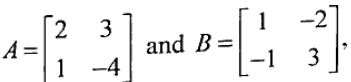then verify that (AB)-1 = B-1A-1.

OR

[b] Prove that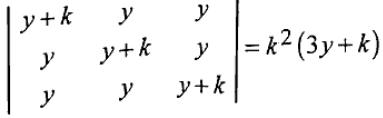.

Solution:

[a]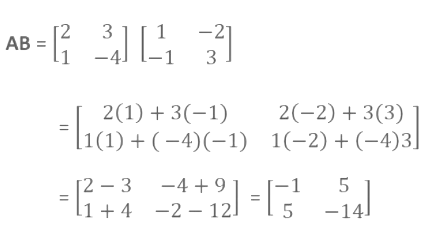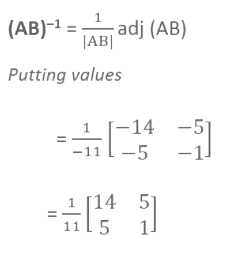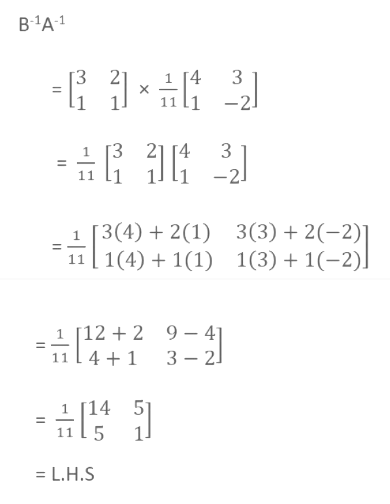LHS = RHS

OR

[b]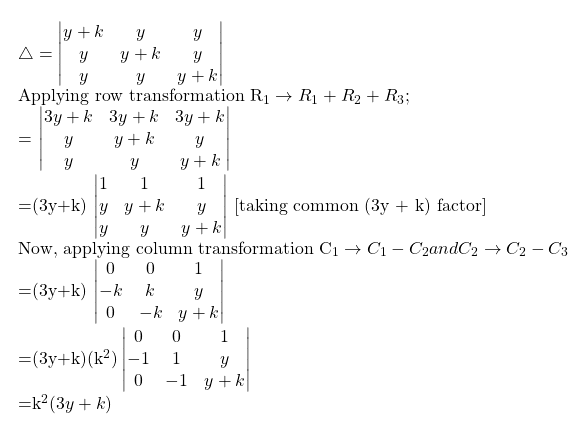Question 18: [a] Find the distance of a point (2, 5, -3) from the plane r · (6i – 3j + 2k) = 4.

OR

[b] Find the vector and cartesian equations of the plane which passes through the point (5, 2, -4) and perpendicular to the line with direction ratios 2, 3, -1.

Solution:

[a] a = 2i + 5j – 3k

N = 6i – 3j + 2k

d = 4

Distance of a point from a plane = |(Ax1 + By1 + Cz1 – D) / √A2 + B2 + C2|

The distance of the point (2, 5 – 3) from the given plane

= |(2i + 5j – 3k) (6i – 3j + 2k) – 4 / |6i – 3j + 2k|

= |12 – 15 – 6 – 4 / √36 + 9 + 4|

= 13 / 7

OR

[b] The vector equation of the plane passing through a point a and normal to n is given by [{r} . {n} = {a} . {n}]

Substituting a = 5i + 2j – 4k and n = 2i + 3j – k (because the direction ratios of n are 2, 3, -1)},

{r} . (2i + 3j – k}) = 5i + 2j – 4k and n. (2i + 3j – k)

{r} . (2i + 3j – k}) = 10 + 6 + 4

{r} . (2i + 3j – k}) = 20

For Cartesian form, substitute r = r . (xi + yj – zk}) in this equation.

Then, (xi + yj + zk) . (2i + 3j – k}) = 20

2x + 3y – z = 20 is the required equation.

Question 19: [a] Solve the following linear programming problem graphically: Maximum : Z = 4x+ y

Subject to the constraints

x + y ≤ 50

3x+ y ≤ 90

x ≥ 0

y ≥ 0

OR

[b] A cooperative society or farmers has 50 hectares of land to grow two crops

X and Y. The profit from crops X and Y per hectare is estimated as

Rs. 10,500 and Rs. 9,000 respectively. To control weeds, a liquid herbicide

has to be used for crops X and Y at rates or 20 litres and 10 litres per hectare.

Further. no more than 800 litres of herbicides should be used in order to

protect fish and wildlife using a pond which collects drainage from this land.

How much land should be allocated to each crop so as to maximise the total

profit of society?

Solution:

[a]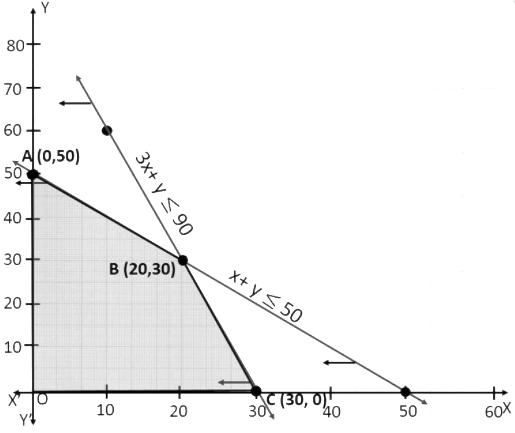Points Z (0, 50) 50 (20, 30) 110 (30, 0) 120 (0, 0) 0

Z is the minimum at (30, 0).

OR

[b] Let x hectare of land be allocated to crop X and Y hectare to crop Y.

Profit per hectare on crop X = Rs 10500

Profit per hectare on crop Y = Rs 9000

Total profit = Rs (10500x + 9000y)

The formulation of the problem is:

Maximise Z = 10500 x + 9000 y

Subject to the constraints:

x + y ≤ 50 (land constraint) … (1)

20x + 10y ≤ 800 (herbicide constraint)

2x + y ≤ 80 … (2)

x ≥ 0, y ≥ 0 (non negative constraint) … (3)

The feasible region is OABC.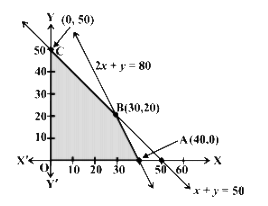Points Z (0, 50) 450000 (30, 20) 495000 (40, 0) 420000 (0, 0) 0

The society will get a maximum profit of Rs 4,95,000 by allocating 30 hectares for crop X and 20 hectares for crop Y.

Question 20: [a] Of the students in a college, it is known that 60% reside in a hostel and 40% are day scholars (not residing in hostel). Previous year results report that 30% of all students who reside in hostel attain A grade and 20% of day scholars attain A grade in their annual examination. At the end of the year, one student is chosen at random from the college and he has an A grade, what is the probability that the student is from the hostel?

OR

[b] An unbiased die is thrown twice. Let the event A be “Odd number on the first throw” and B the event “Odd number on the second throw”. Check the independence of the events A and B.

Solution:

[a] Let A, E1 and E2 denote the events that the selected student attains grade A, resides in a hostel and does not reside in a hostel, respectively.

P ( E1 ) = {60} / {100}

P ( E2 ) = {40} / {100}

P ( A / E1 ) = {30} / {100}

P ( A / E2 ) = {20} / {100}

Using Bayes’ theorem,

Required probability = P ( E1 / A ) = {P ( E1 ) ( A / E1 )} / {P ( E1 ) ( A / E1 )} + P ( E2 ) ( A / E2 )}}

= {{60} / {100} * {30} / {100}} / {{60} / {100} * {30} / {100} + {40} / {100} * {20} / {100}}

= {18} / {18 + 8}

= {9} / {13}

OR

[b] Any two events A and B are said to be independent if P(A ∩ B) = P(A) . P(B)

An unbiased die is thrown twice.

The sample space is given by

S = {(1, 1), (1, 2), (1, 3), (1, 4), (1, 5), (1, 6),

(2, 1), (2, 2), (2, 3), (2, 4), (2, 5), (2, 6),

(3, 1), (3, 2), (3, 3), (3, 4), (3, 5), (3, 6),

(4, 1), (4, 2), (4, 3), (4, 4), (4, 5), (4, 6),

(5, 1), (5, 2), (5, 3), (5, 4), (5, 5), (5, 6),

(6, 1), (6, 2), (6, 3), (6, 4), (6, 5), (6, 6)}

Let A : Odd number on the First throw

B : Odd number on the Second throw

A : Odd number on the first throw

A : { (1, 1), (1, 2), ………., (1, 6)

(3, 1), (3, 2), ………., (3, 6)

(5, 1), (5, 2), ………., (5, 6) }

P (A) = 18 / 36 = 1 / 2

B : Odd number on the second throw

B : { (1, 1), (2, 1), ………., (6, 1)

(1, 3), (2, 3), ………., (6, 3)

(1, 5), (2, 5), ………., (6, 5) }

P (B) = 18 / 36 = 1 / 2

A ∩ B = Odd number on the first and second throw

= { (1, 1), (1, 3), (1, 5), (3, 1), (3, 3), (3, 5), (5, 1), (5, 3), (5, 5)}

P(A ∩ B) = 9 / 36 = 1 / 4

P (A) . P (B) = 1 / 2 × 1 / 2 = 1 / 4

P(A ∩ B) = P(A) . P(B)

So, A and B are independent events.

Question 21: [a] From a lot of 30 bulbs which include 6 defectives, a sample of 4 bulbs is drawn at random with replacement. Find the probability distribution of the number of defective bulbs.

OR

[b] Ten cards numbered 1 to 10 are placed in a box, mixed up thoroughly and then one card is drawn randomly. If it is known that the number on the drawn card is more than 3, what is the probability that it is an even number?

Solution:

[a] Total bulbs = 30

Defective bulbs = 6

Non defective bulbs = 30 – 6 = 24

P (defective bulbs) ={6} / {30} = {1} / {5}

P (non defective bulbs) = {24} / {30} = {4} / {5}

4 bulbs are drawn at random with replacement.

Let X : number of defective bulbs

P (X = 0) = 4C0{4} / {5}.{4} / {5}.{4} / {5}.{4} / {5} = {256} / {625}

P(X = 1) = 4C1{1} / {5}.{4} / {5}.{4} / {5}.{4} / {5} = {256} / {625}

P(X = 2) = 4C2 {1} / {5}.{1} / {5}.{4} / {5}.{4} / {5} = {96} / {625}

P(X = 3) = 4C3 {1} / {5}.{1} / {5}.{1} / {5}.{4} / {5} = {16} / {625}

P(X = 4) = 4C4 {1} / {5}.{1} / {5}.{1} / {5}.{1} / {5} = {1} / {625}

The probability distribution of the number of defective bulbs is as :

 X 0 1 2 3 4 P(X) {256} / {625} {256} / {625} {96} / {625} {16} / {625} {1} / {625}

OR

[b] Total number of cards in the box n (S) = {1, 2, 3, 4, 5, 6, 7, 8, 9, 10) = 10.

(i) Let A be the event of drawing a card which is an even number.

=> n (A) = {2, 4, 6, 8, 10}

= 5

Required probability P (A) = n (A) / n (S) = 5 / 10

(ii) Let B be the event of drawing a number that is more than 3.

=> n (B) = {4, 5, 6, 7, 8, 9, 10}

= 7

The required probability P (B) = n (B) / n (S) = 7 / 10

(A ∩ B) = {4, 6, 8, 10} = 4 / 10

P (A / B) = P (A ∩ B) / P (B)

= (4 / 10) * (10 / 7)

= (4 / 7)

Question 22: [a] Express the matrix B =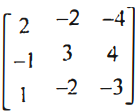as the sum of a symmetric and a skew-symmetric matrix.

OR

[b] By using elementary operations, find the inverse of the matrix A =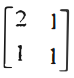.

Solution:

[a]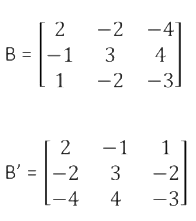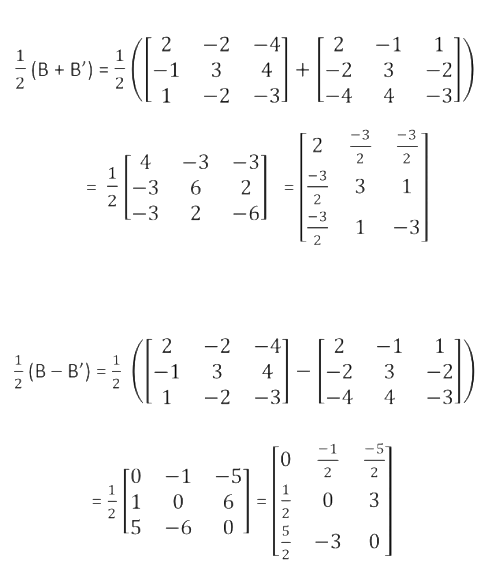Let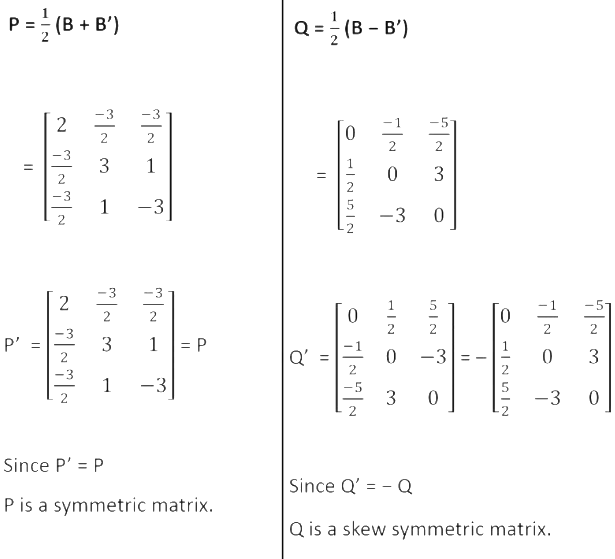P + Q = (1 / 2) [B + B’] + (1 / 2) (B – B’)

= B

Thus B is a sum of a symmetric and skew-symmetric matrix.

OR

[b]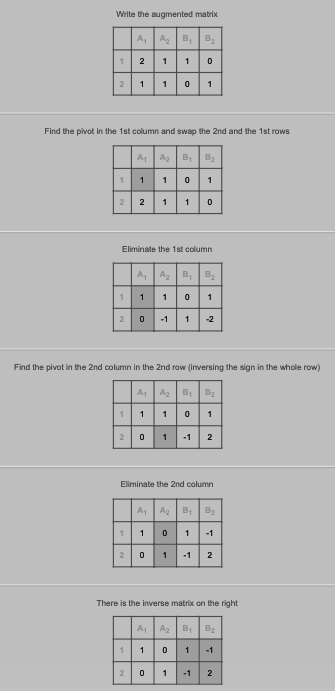Question 23: [a] Verify Rolle’s theorem for the function f (x) = x2 + 2x – 8, x ∈ [- 4, 2].

OR

[b] Differentiate ax wrt x, where a is a positive constant.

Solution:

[a] f (x) is a polynomial in x.

Hence it is continuous over [-4, 2] and differentiable over (-4, 2).

f (- 4) = (- 4)2 + 2 (- 4) – 8

= 16 – 8 – 8

= 0

f (2) = 4 + 4 – 8 = 0

∴ f (- 4) = f (2)

∴ All the conditions of the Rolle’s theorem are satisfied.

There exists a c ∈ [- 4, 2] such that f ‘ (c) = 0

f ‘ (x) = 2x + 2

f (c) = 2c + 2

f (c) = 0

⇒ 2c + 2 = 0

⇒ 2c = -2

⇒ c = – 1 ∈ [- 4, 2]

∴ Rolle’s theorem is verified.

OR

[b] Let y = 𝑎𝑥

Taking log on both sides

log⁡ 𝑦 = log⁡ 𝑎𝑥

log⁡ 𝑦 = 𝑥 log⁡ 𝑎

Differentiating both sides with respect to x,

(𝑑 (log⁡ 𝑦)) / 𝑑𝑥 = 𝑑 / 𝑑𝑥 (𝑥 log⁡𝑎)

(𝑑 (log⁡ 𝑦)) / 𝑑𝑥 = log⁡𝑎 (𝑑𝑥 / 𝑑𝑥)

(𝑑 (log⁡𝑦)) / 𝑑𝑥 = log⁡𝑎

(𝑙𝑜𝑔⁡ 𝑎𝑏 = 𝑏 𝑙𝑜𝑔⁡𝑎)

(𝑑 (log⁡𝑦)) / 𝑑𝑥 . 𝑑𝑦 / 𝑑𝑦 = log⁡𝑎

(𝑑(log⁡𝑦)) / 𝑑𝑦 . 𝑑𝑦 / 𝑑𝑥 = log⁡𝑎

(1 / 𝑦) . 𝑑𝑦 / 𝑑𝑥 = log⁡𝑎

𝑑𝑦 / 𝑑𝑥 = 𝑦 log⁡𝑎

Putting back 𝑦 = 𝑎𝑥

𝒅𝒚 / 𝒅𝒙 = 𝒂𝒙 𝒍𝒐𝒈⁡𝒂

Question 24: [a] Evaluate ∫0𝛑/2 sin4 x / [sin4 x + cos4 x] dx.

OR

[b] Evaluate ∫(x2 + 1) ex / (x + 1)2 dx.

Solution:

[a] I = ∫0𝛑/2 sin4 x / [sin4 x + cos4 x] dx — (1)

= ∫0𝛑/2 sin4 (𝛑 / 2 – x) / [sin4 (𝛑 / 2 – x) + cos4 (𝛑 / 2 – x)] dx

= ∫0𝛑/2 cos4 x / [sin4 x + cos4 x] dx —- (2)

I + I = ∫0𝛑/2 sin4 x / [sin4 x + cos4 x] dx + ∫0𝛑/2 cos x / [sin4 x + cos4 x] dx

2I = ∫0𝛑/2 {[sin4 x + cos4 x] / [sin4 x + cos4 x]} dx

2I = ∫0𝛑/2 dx

2I = [𝛑 / 2 – 0]

I = 𝛑 / 4

OR

[b]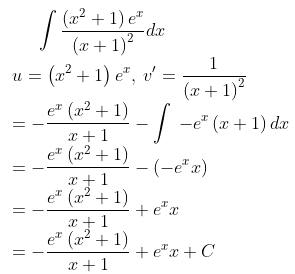Question 25: [a] Using integration, find the area of the region bounded by the triangle whose vertices are (1, 0), (2, 2) and (3, 1).

OR

[b] Find the area of the parabola y2 = 4ax bounded by its latus rectum.

Solution:

[a]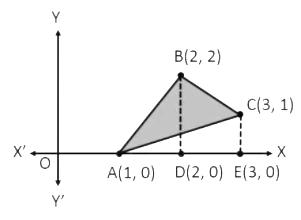Area of triangle ABD = ∫12 y dx

= ∫12 2 (x – 1) dx

= 2 [x2 / 2 – x]12

= 2 [2 – 2 – (1 / 2) + 1]

= 2 [1 / 2]

= 1

Area of BDEC

= ∫23 y dx

= ∫23 (4 – x) dx

= 4 ∫23dx – ∫23 x dx

= 4 [x]23 – [x2 / 2]23

= 4 – (5 / 2)

= 3 / 2

Area of triangle ACE = ∫13 y dx

= ∫13 (1 / 2) (x – 1) dx

= 1 / 2 [x2 / 2 – x]13

= 1 / 2 [9 / 2 – 3 – (1 / 2) + 1]

= 1 / 2 [4 / 2]

= 1

Required area = Area of ABD + Area of BDEC – Area of ACE

= 1 + (3 / 2) – 1

= 3 / 2

OR

[b] Given equation of parabola is: y2 = 4ax

y = √(4ax) = 2√a √x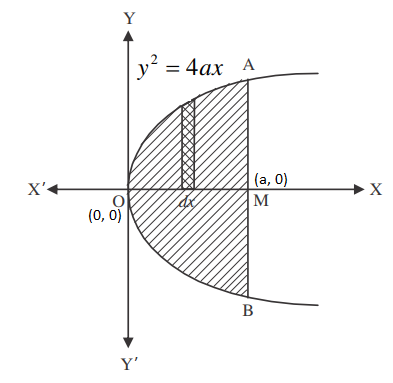Required area = Area of AOBMA

= 2 × Area of AOMA

= 2 ∫0a y dx

= 2 ∫0a 2 √a √x dx

= 4√a ∫0a (x)1/2 dx

= 4√a [x3/2 / (3 / 2)]0a

= 4√a × (2 / 3) × [(a)3/2 – 0]

= (8√a / 3) × (a3/2)

= 8a2 / 3 square units

Question 26: [a] Solve the differential equation dy / dx + y / x = x2.

OR

[b] Find the particular solution of the differential equation log (dy / dx) = 3x + 4y, given that y = 0 when x = 0.

Solution:

[a] dy / dx + y / x = x2

This differential equation is of the form (dy / dx) + Py = Q.

P = (1 / x)

Q = x2

IF = e∫P dx

= e∫(1/x) dx

= elogx

= x

The solution is

y (IF) = ∫(Q * IF) dx + c

yx = ∫x2 * x * dx + c

xy = x4 / 4 + c

OR

[b] log (𝑑𝑦 / 𝑑𝑥) = 3𝑥 + 4𝑦

𝑑𝑦 / 𝑑𝑥 = e(3x + 4y)

𝑑𝑦 / 𝑑𝑥 = e3x e4y

Separating the variables,

𝑑𝑦 / 𝑒4𝑦 = e3x dx

e−4y dy = e3x dx

Integrating both sides

∫𝑒(−4𝑦) 𝑑𝑦 = ∫𝑒3𝑥 𝑑𝑥

𝑒(−4𝑦) / (−4) = 𝑒3𝑥 / 3 + 𝐶

0 = 𝑒3𝑥 / 3 + 𝑒(−4𝑦) / 4 + 𝐶

𝑒^3𝑥/3 + 𝑒^(−4𝑦)/4 + C = 0

(4𝑒3𝑥 + 3𝑒(−4𝑦)) / 12 + C = 0

(4𝑒3𝑥 + 3𝑒(−4𝑦) + 12𝐶) / 12 = 0

4𝑒3𝑥 + 3𝑒(−4𝑦) + 12C = 0

Given y = 0 when x = 0…(1)

Putting x = 0 y = 0 in (1)

4𝑒(3(0)) + 3𝑒(−4(0)) + 12C = 0

4𝑒0 + 3𝑒0 + 12C = 0

4 + 3 + 12C = 0

7 + 12C = 0

12C = – 7

C = (−7) / 12

Putting value of C in (1)

4𝑒3𝑥 + 3𝑒(−4𝑦) + 12C = 0

4𝑒3𝑥 + 3𝑒(−4𝑦) + 12 ((−7) / 12) = 0

𝟒𝒆𝟑𝒙 + 𝟑𝒆(−𝟒𝒚) − 7 = 0